Скачать презентацию Practical Statistical Relational AI Pedro Domingos Dept of

bc97ba5c3521c14c329142d1fc080d6c.ppt

• Количество слайдов: 88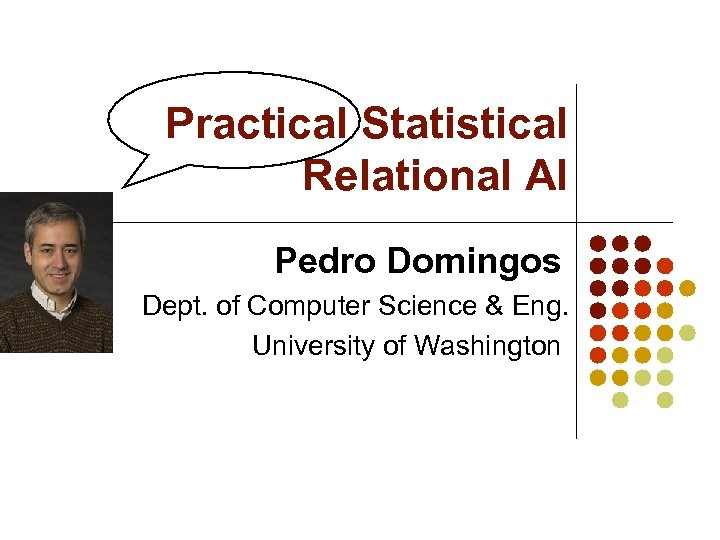Practical Statistical Relational AI Pedro Domingos Dept. of Computer Science & Eng. University of Washington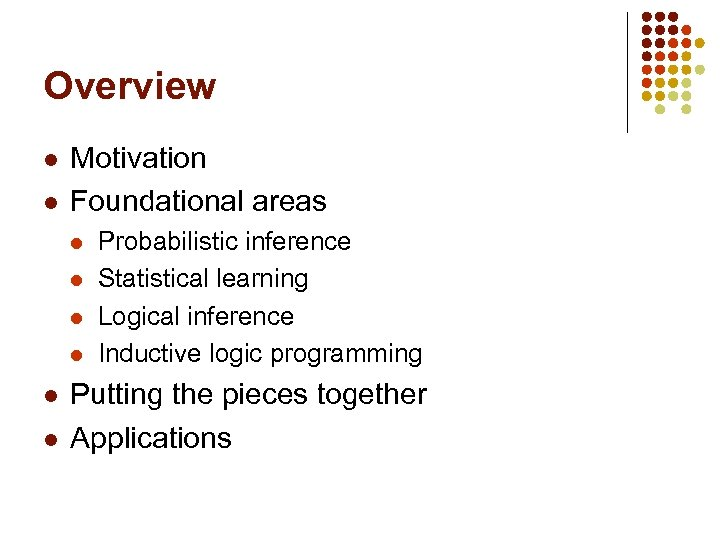Overview l l Motivation Foundational areas l l l Probabilistic inference Statistical learning Logical inference Inductive logic programming Putting the pieces together Applications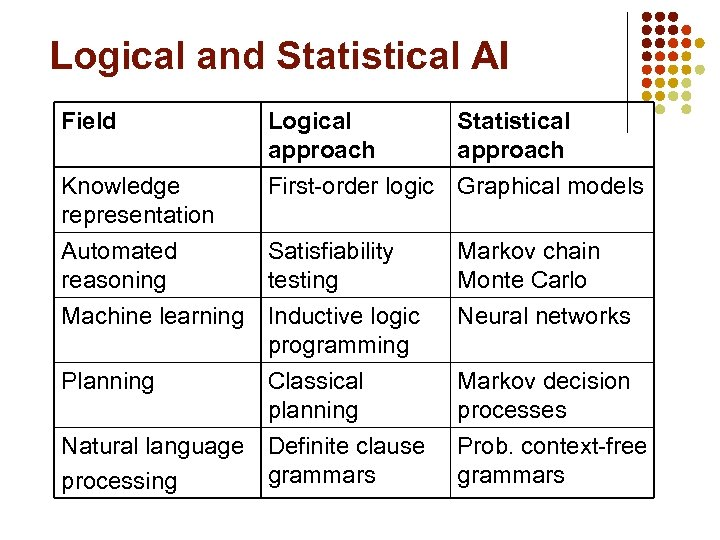Logical and Statistical AI Field Logical approach Statistical approach Knowledge representation First-order logic Graphical models Automated reasoning Satisfiability testing Markov chain Monte Carlo Machine learning Inductive logic programming Neural networks Planning Markov decision processes Classical planning Natural language Definite clause grammars processing Prob. context-free grammars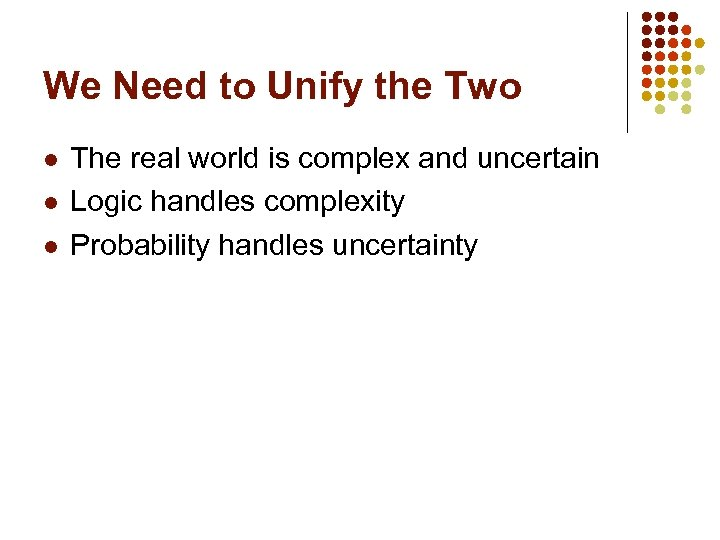We Need to Unify the Two l l l The real world is complex and uncertain Logic handles complexity Probability handles uncertainty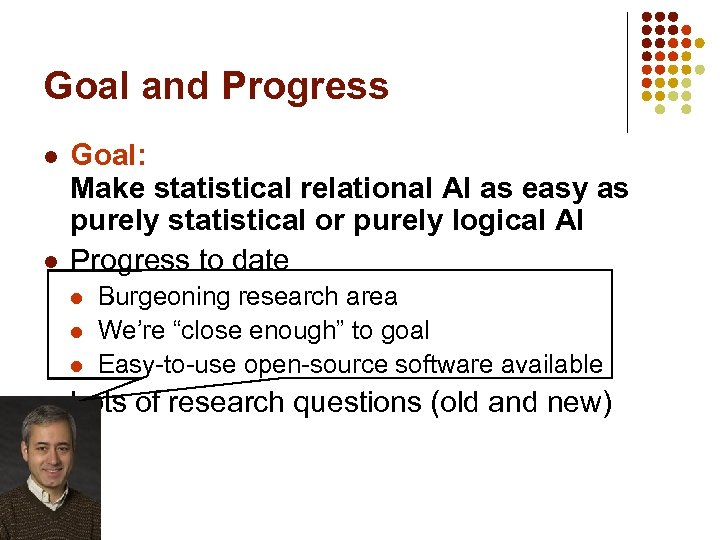Goal and Progress l l Goal: Make statistical relational AI as easy as purely statistical or purely logical AI Progress to date l l Burgeoning research area We’re “close enough” to goal Easy-to-use open-source software available Lots of research questions (old and new)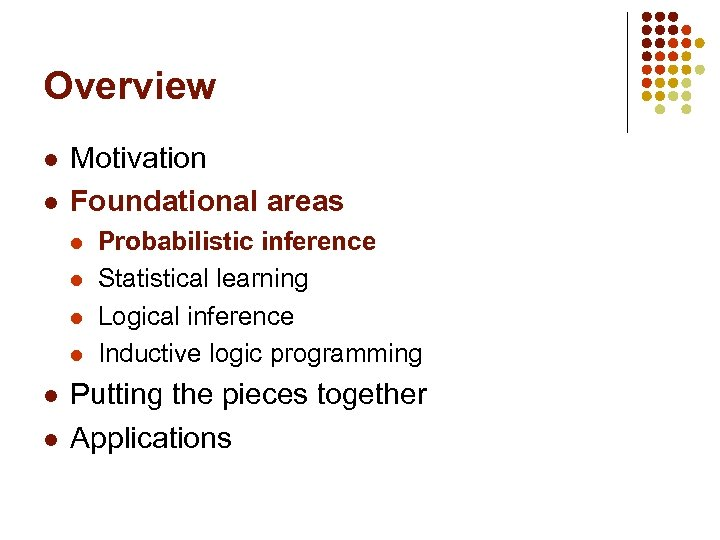Overview l l Motivation Foundational areas l l l Probabilistic inference Statistical learning Logical inference Inductive logic programming Putting the pieces together Applications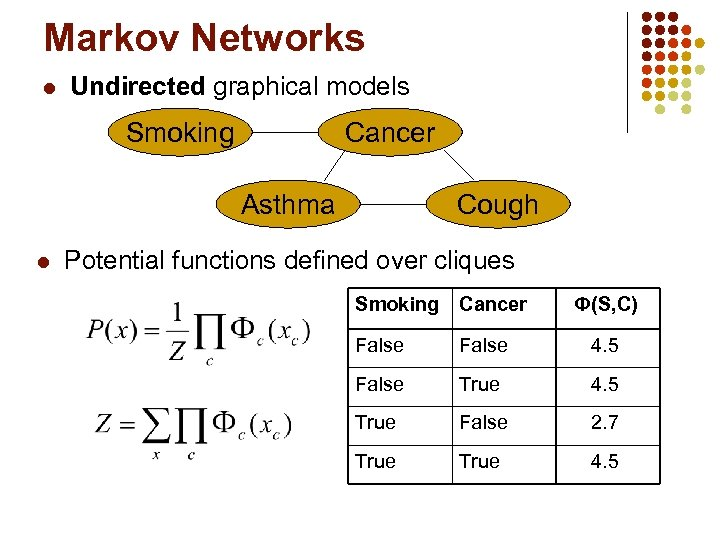Markov Networks l Undirected graphical models Smoking Cancer Asthma l Cough Potential functions defined over cliques Smoking Cancer Ф(S, C) False 4. 5 False True 4. 5 True False 2. 7 True 4. 5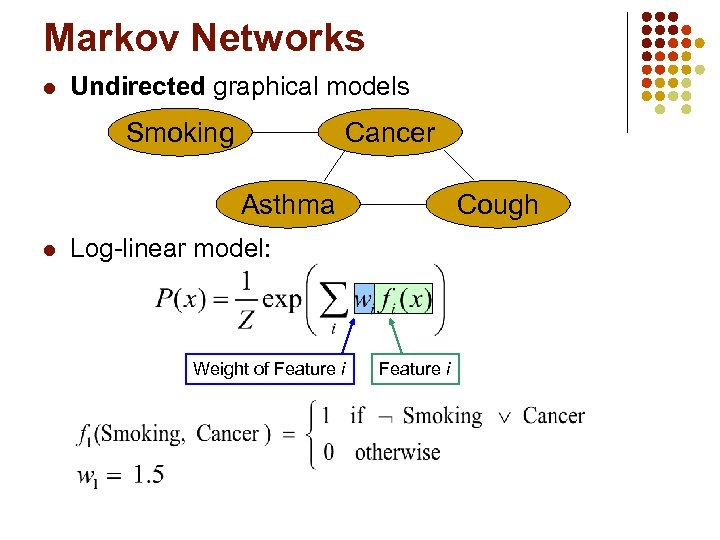Markov Networks l Undirected graphical models Smoking Cancer Asthma l Cough Log-linear model: Weight of Feature i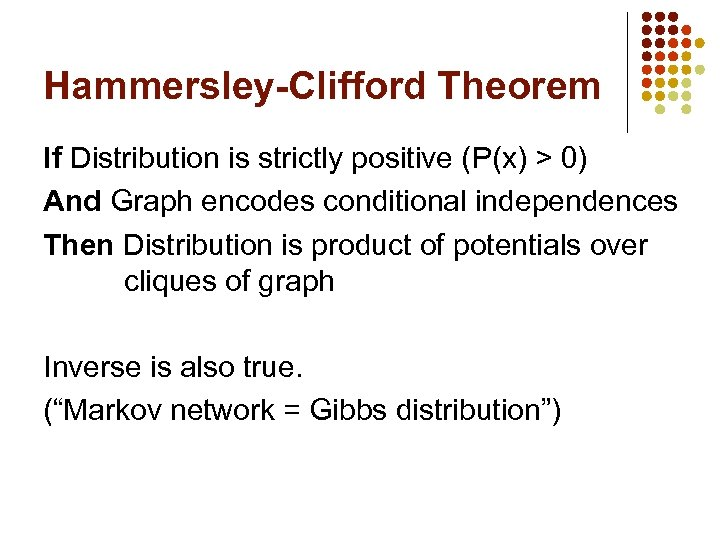Hammersley-Clifford Theorem If Distribution is strictly positive (P(x) > 0) And Graph encodes conditional independences Then Distribution is product of potentials over cliques of graph Inverse is also true. (“Markov network = Gibbs distribution”)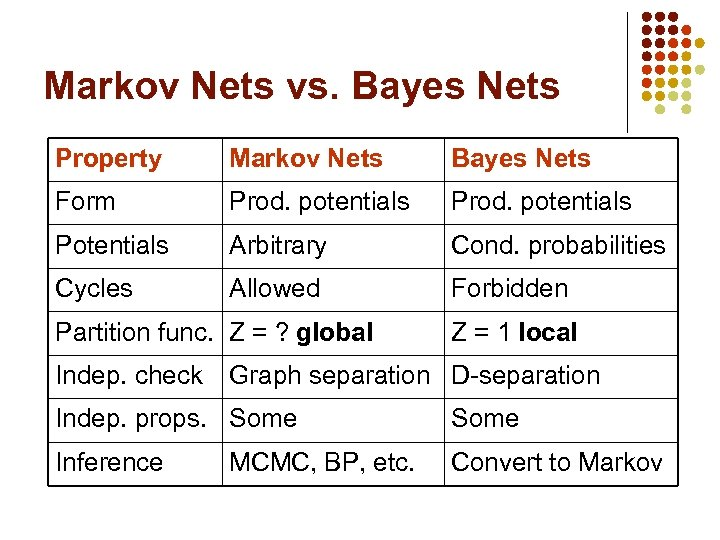Markov Nets vs. Bayes Nets Property Markov Nets Bayes Nets Form Prod. potentials Potentials Arbitrary Cond. probabilities Cycles Allowed Forbidden Partition func. Z = ? global Indep. check Z = 1 local Graph separation D-separation Indep. props. Some Inference Convert to Markov MCMC, BP, etc.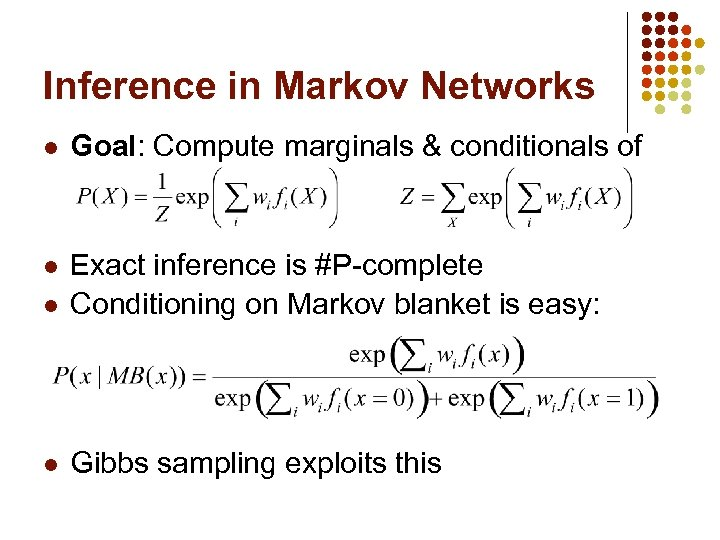Inference in Markov Networks l Goal: Compute marginals & conditionals of l l Exact inference is #P-complete Conditioning on Markov blanket is easy: l Gibbs sampling exploits this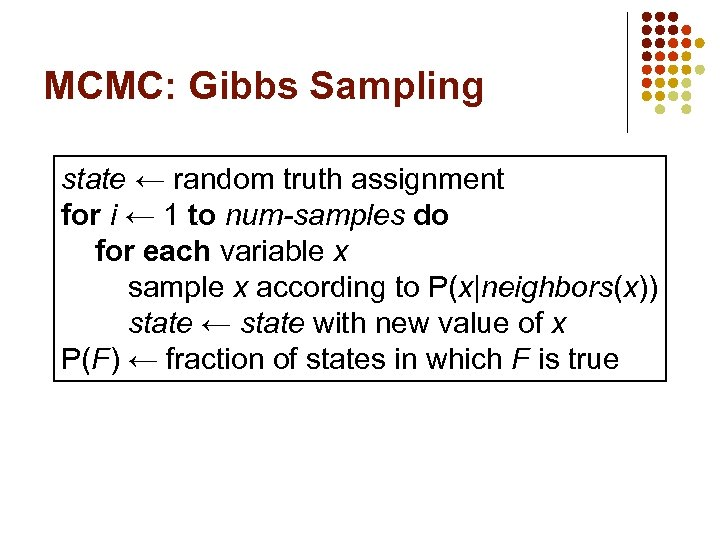MCMC: Gibbs Sampling state ← random truth assignment for i ← 1 to num-samples do for each variable x sample x according to P(x|neighbors(x)) state ← state with new value of x P(F) ← fraction of states in which F is true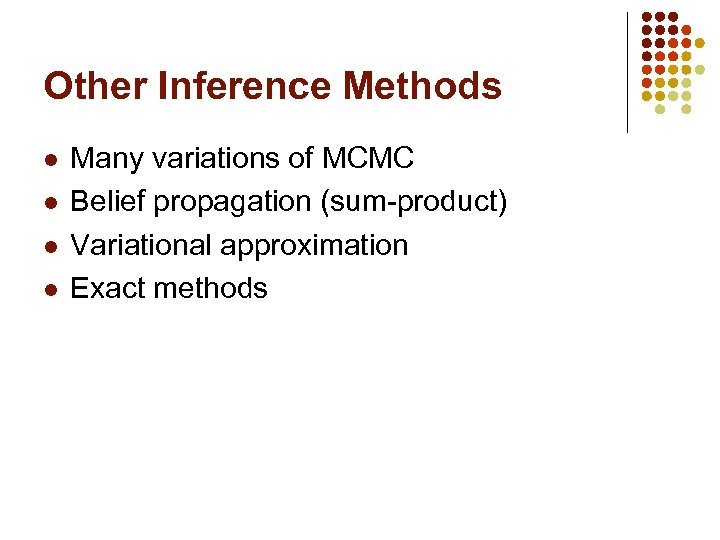Other Inference Methods l l Many variations of MCMC Belief propagation (sum-product) Variational approximation Exact methods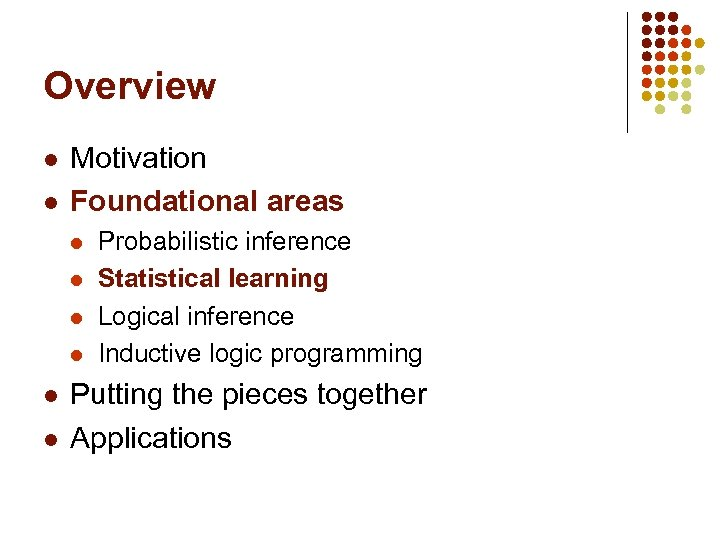Overview l l Motivation Foundational areas l l l Probabilistic inference Statistical learning Logical inference Inductive logic programming Putting the pieces together Applications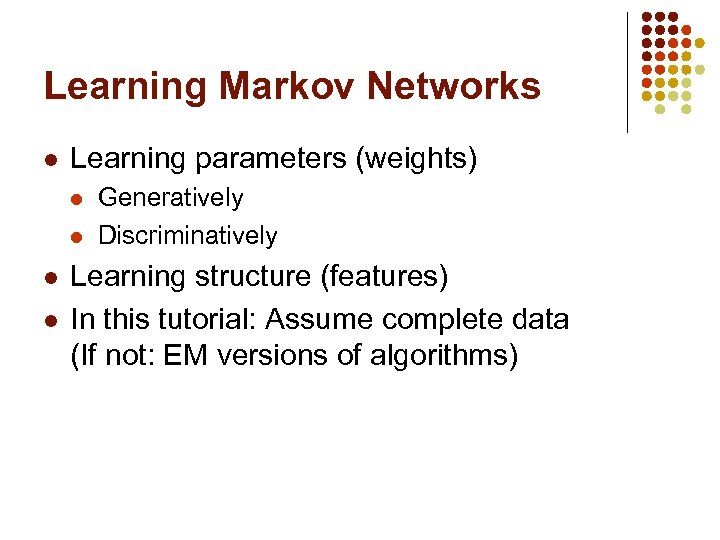Learning Markov Networks l Learning parameters (weights) l l Generatively Discriminatively Learning structure (features) In this tutorial: Assume complete data (If not: EM versions of algorithms)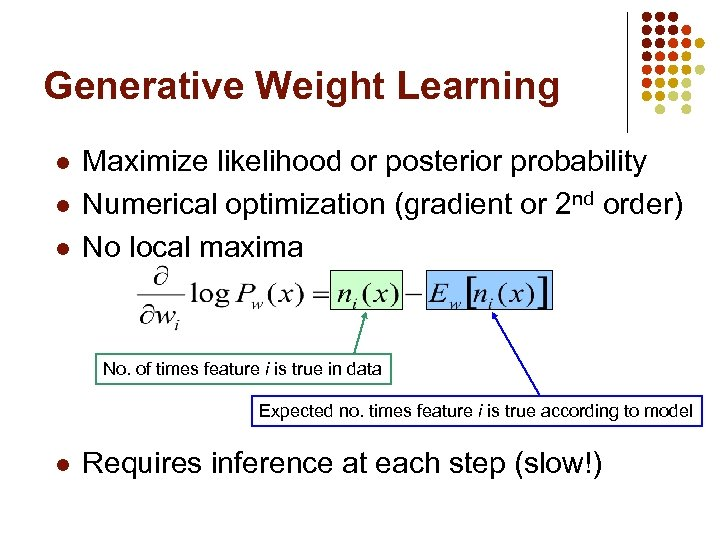Generative Weight Learning l l l Maximize likelihood or posterior probability Numerical optimization (gradient or 2 nd order) No local maxima No. of times feature i is true in data Expected no. times feature i is true according to model l Requires inference at each step (slow!)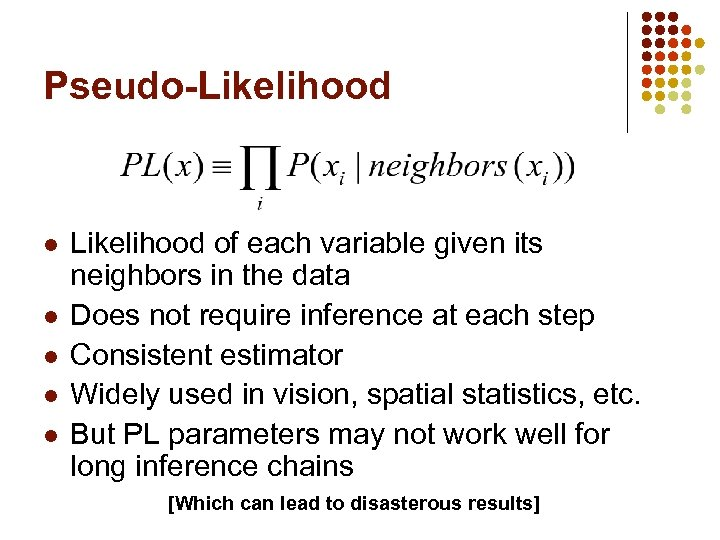Pseudo-Likelihood l l l Likelihood of each variable given its neighbors in the data Does not require inference at each step Consistent estimator Widely used in vision, spatial statistics, etc. But PL parameters may not work well for long inference chains [Which can lead to disasterous results]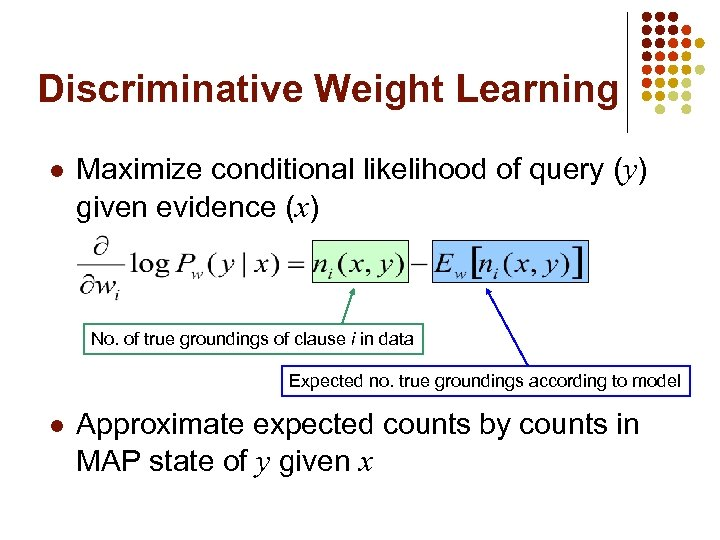Discriminative Weight Learning l Maximize conditional likelihood of query (y) given evidence (x) No. of true groundings of clause i in data Expected no. true groundings according to model l Approximate expected counts by counts in MAP state of y given x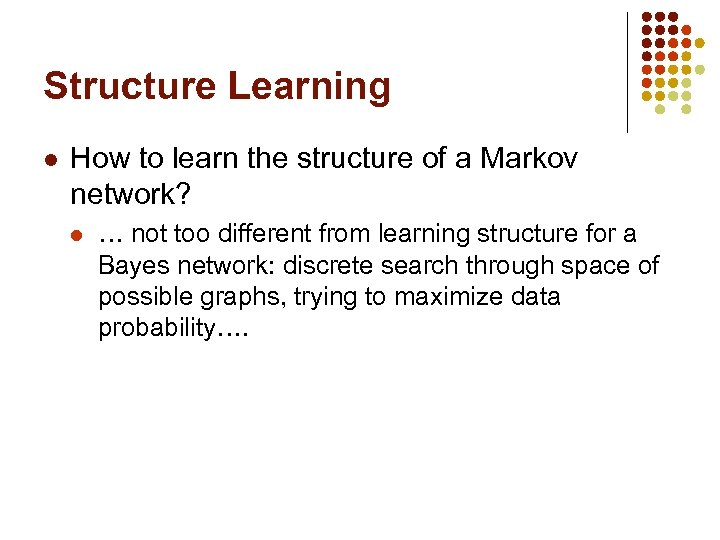Structure Learning l How to learn the structure of a Markov network? l … not too different from learning structure for a Bayes network: discrete search through space of possible graphs, trying to maximize data probability….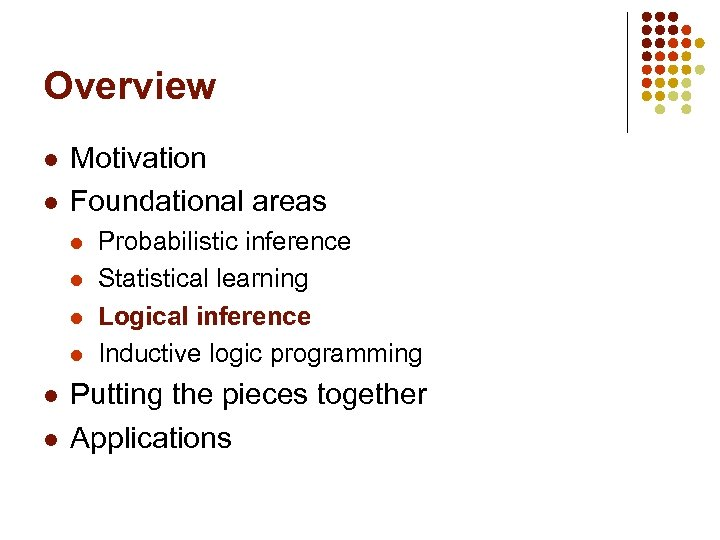Overview l l Motivation Foundational areas l l l Probabilistic inference Statistical learning Logical inference Inductive logic programming Putting the pieces together Applications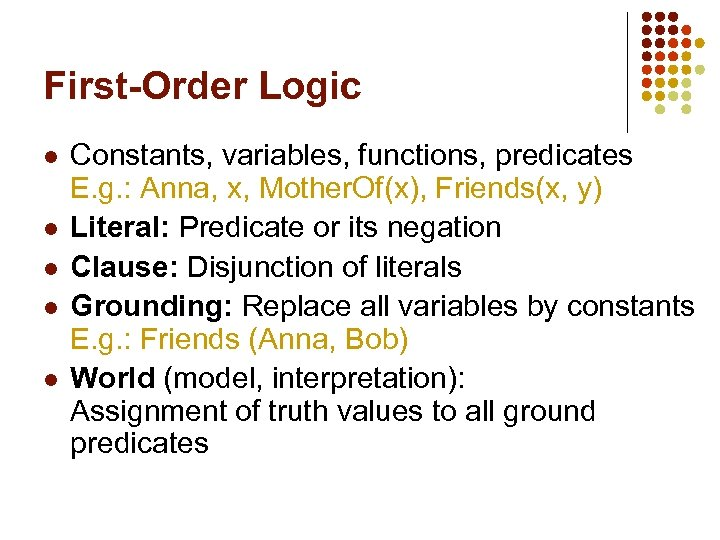First-Order Logic l l l Constants, variables, functions, predicates E. g. : Anna, x, Mother. Of(x), Friends(x, y) Literal: Predicate or its negation Clause: Disjunction of literals Grounding: Replace all variables by constants E. g. : Friends (Anna, Bob) World (model, interpretation): Assignment of truth values to all ground predicates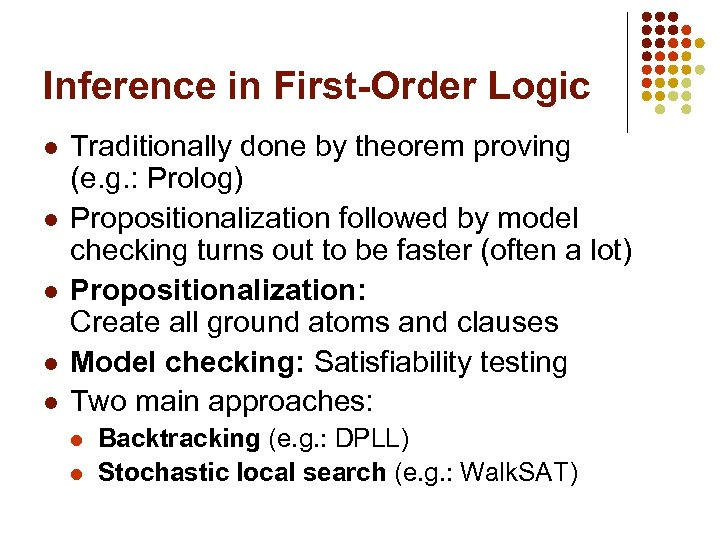Inference in First-Order Logic l l l Traditionally done by theorem proving (e. g. : Prolog) Propositionalization followed by model checking turns out to be faster (often a lot) Propositionalization: Create all ground atoms and clauses Model checking: Satisfiability testing Two main approaches: l l Backtracking (e. g. : DPLL) Stochastic local search (e. g. : Walk. SAT)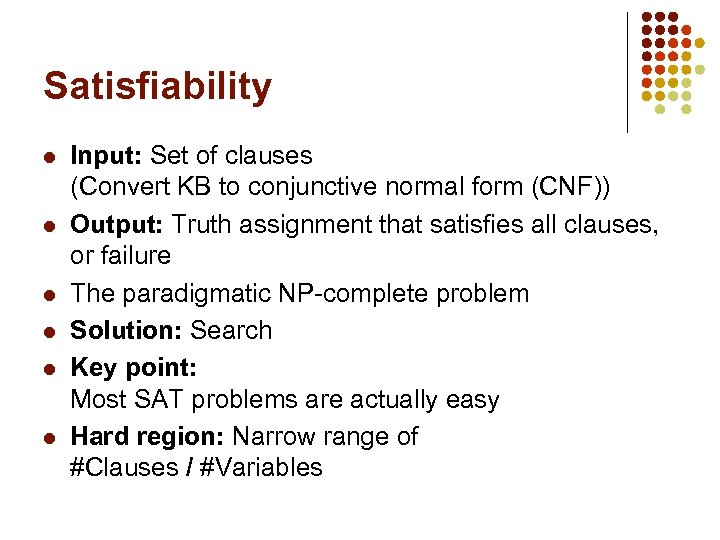Satisfiability l l l Input: Set of clauses (Convert KB to conjunctive normal form (CNF)) Output: Truth assignment that satisfies all clauses, or failure The paradigmatic NP-complete problem Solution: Search Key point: Most SAT problems are actually easy Hard region: Narrow range of #Clauses / #Variables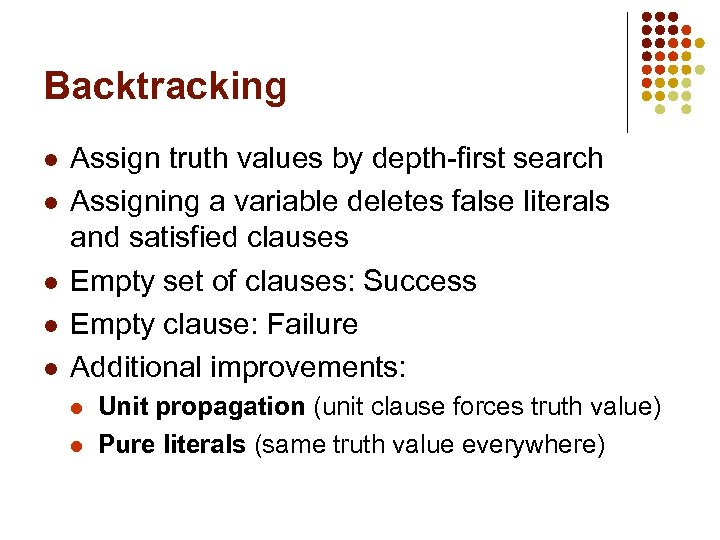Backtracking l l l Assign truth values by depth-first search Assigning a variable deletes false literals and satisfied clauses Empty set of clauses: Success Empty clause: Failure Additional improvements: l l Unit propagation (unit clause forces truth value) Pure literals (same truth value everywhere)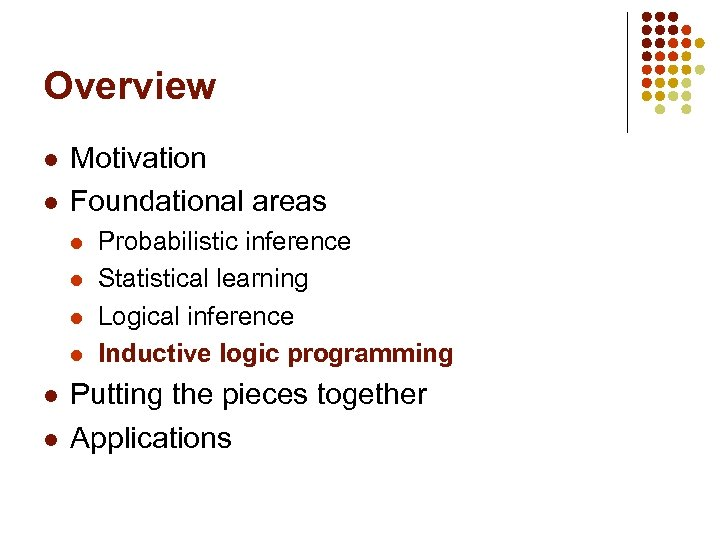Overview l l Motivation Foundational areas l l l Probabilistic inference Statistical learning Logical inference Inductive logic programming Putting the pieces together Applications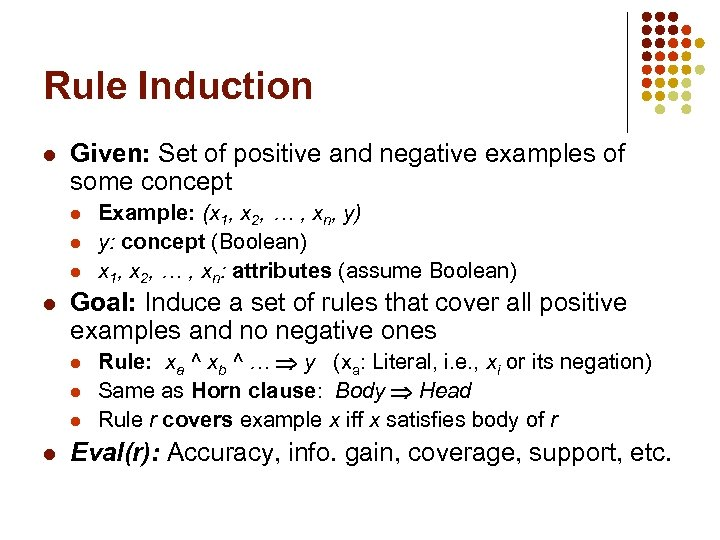Rule Induction l Given: Set of positive and negative examples of some concept l l Goal: Induce a set of rules that cover all positive examples and no negative ones l l Example: (x 1, x 2, … , xn, y) y: concept (Boolean) x 1, x 2, … , xn: attributes (assume Boolean) Rule: xa ^ xb ^ … y (xa: Literal, i. e. , xi or its negation) Same as Horn clause: Body Head Rule r covers example x iff x satisfies body of r Eval(r): Accuracy, info. gain, coverage, support, etc.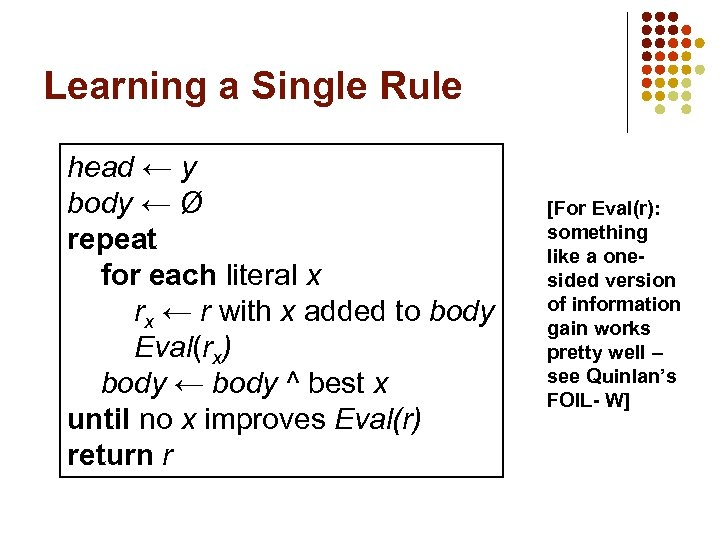Learning a Single Rule head ← y body ← Ø repeat for each literal x rx ← r with x added to body Eval(rx) body ← body ^ best x until no x improves Eval(r) return r [For Eval(r): something like a onesided version of information gain works pretty well – see Quinlan’s FOIL- W]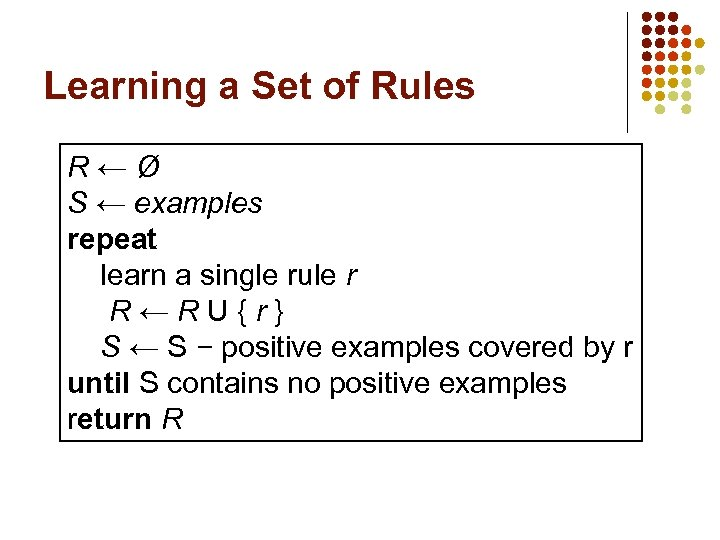Learning a Set of Rules R←Ø S ← examples repeat learn a single rule r R←RU{r} S ← S − positive examples covered by r until S contains no positive examples return R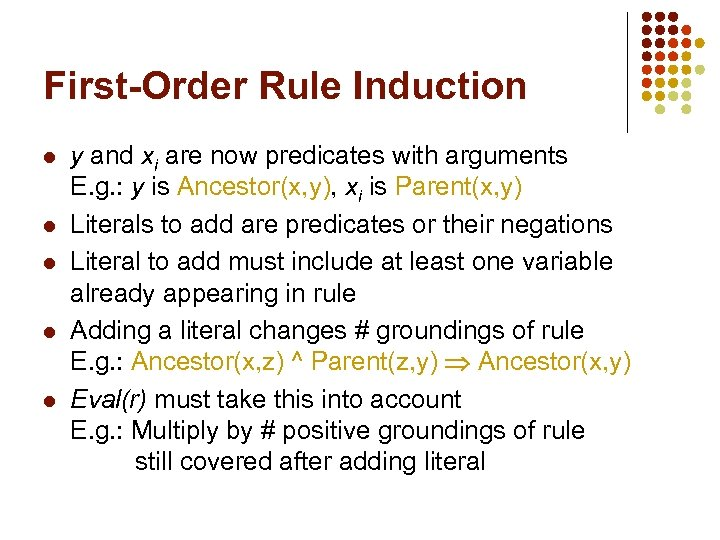First-Order Rule Induction l l l y and xi are now predicates with arguments E. g. : y is Ancestor(x, y), xi is Parent(x, y) Literals to add are predicates or their negations Literal to add must include at least one variable already appearing in rule Adding a literal changes # groundings of rule E. g. : Ancestor(x, z) ^ Parent(z, y) Ancestor(x, y) Eval(r) must take this into account E. g. : Multiply by # positive groundings of rule still covered after adding literal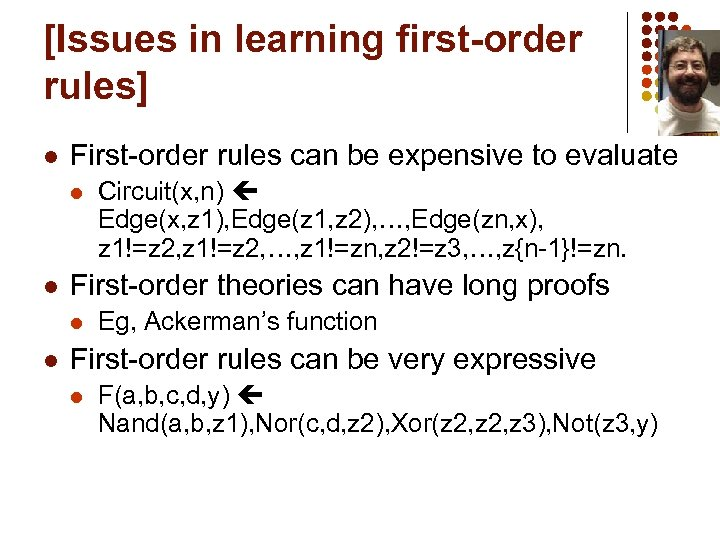[Issues in learning first-order rules] l First-order rules can be expensive to evaluate l l First-order theories can have long proofs l l Circuit(x, n) Edge(x, z 1), Edge(z 1, z 2), …, Edge(zn, x), z 1!=z 2, …, z 1!=zn, z 2!=z 3, …, z{n-1}!=zn. Eg, Ackerman’s function First-order rules can be very expressive l F(a, b, c, d, y) Nand(a, b, z 1), Nor(c, d, z 2), Xor(z 2, z 3), Not(z 3, y)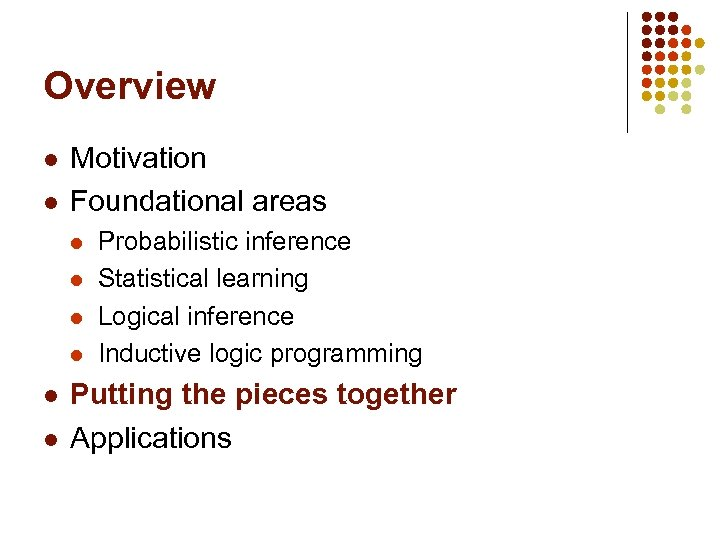Overview l l Motivation Foundational areas l l l Probabilistic inference Statistical learning Logical inference Inductive logic programming Putting the pieces together Applications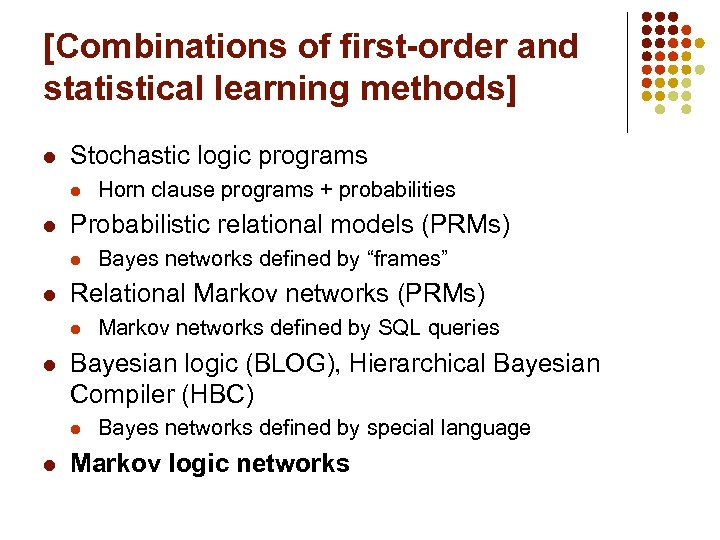[Combinations of first-order and statistical learning methods] l Stochastic logic programs l l Probabilistic relational models (PRMs) l l Markov networks defined by SQL queries Bayesian logic (BLOG), Hierarchical Bayesian Compiler (HBC) l l Bayes networks defined by “frames” Relational Markov networks (PRMs) l l Horn clause programs + probabilities Bayes networks defined by special language Markov logic networks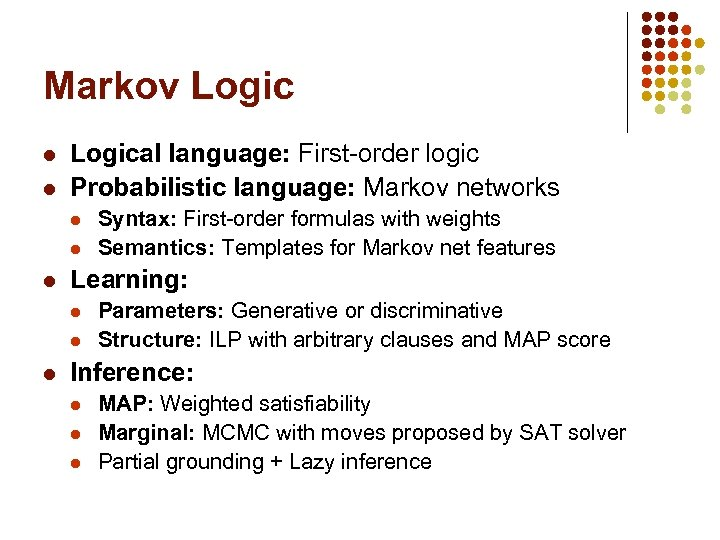Markov Logic l l Logical language: First-order logic Probabilistic language: Markov networks l l l Learning: l l l Syntax: First-order formulas with weights Semantics: Templates for Markov net features Parameters: Generative or discriminative Structure: ILP with arbitrary clauses and MAP score Inference: l l l MAP: Weighted satisfiability Marginal: MCMC with moves proposed by SAT solver Partial grounding + Lazy inference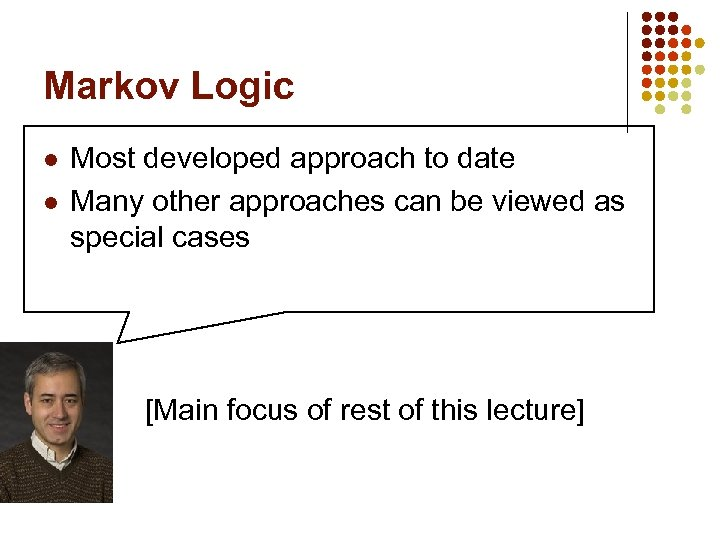Markov Logic l l l Most developed approach to date Many other approaches can be viewed as special cases [Main focus of rest of this lecture]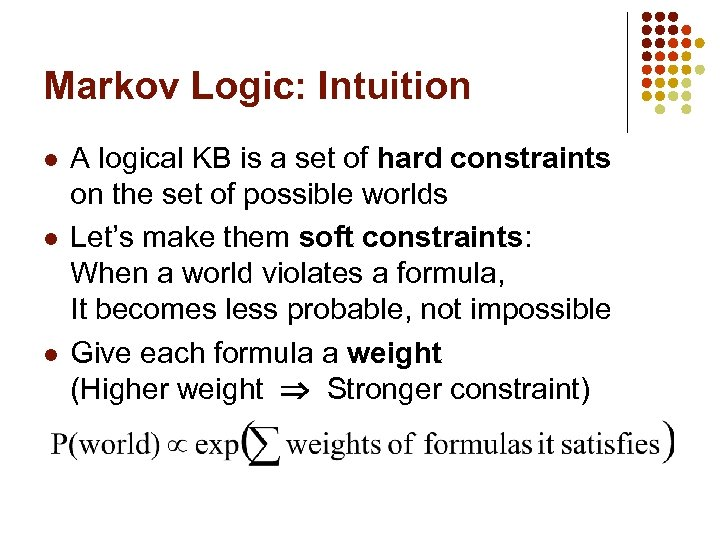Markov Logic: Intuition l l l A logical KB is a set of hard constraints on the set of possible worlds Let’s make them soft constraints: When a world violates a formula, It becomes less probable, not impossible Give each formula a weight (Higher weight Stronger constraint)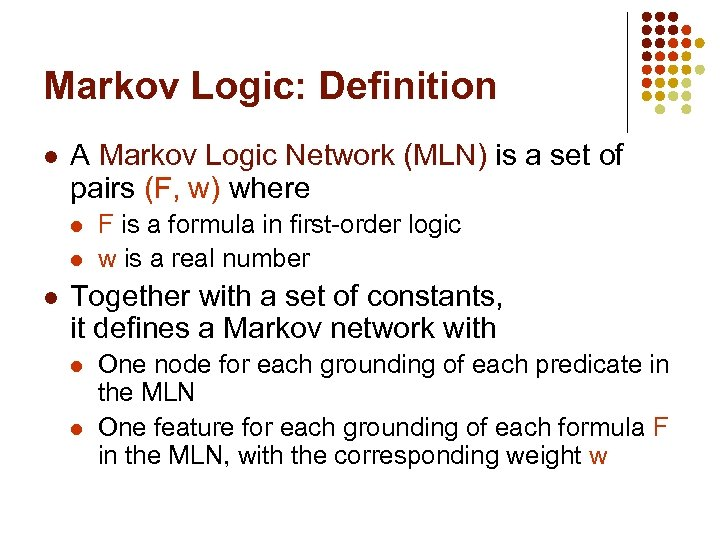Markov Logic: Definition l A Markov Logic Network (MLN) is a set of pairs (F, w) where l l l F is a formula in first-order logic w is a real number Together with a set of constants, it defines a Markov network with l l One node for each grounding of each predicate in the MLN One feature for each grounding of each formula F in the MLN, with the corresponding weight w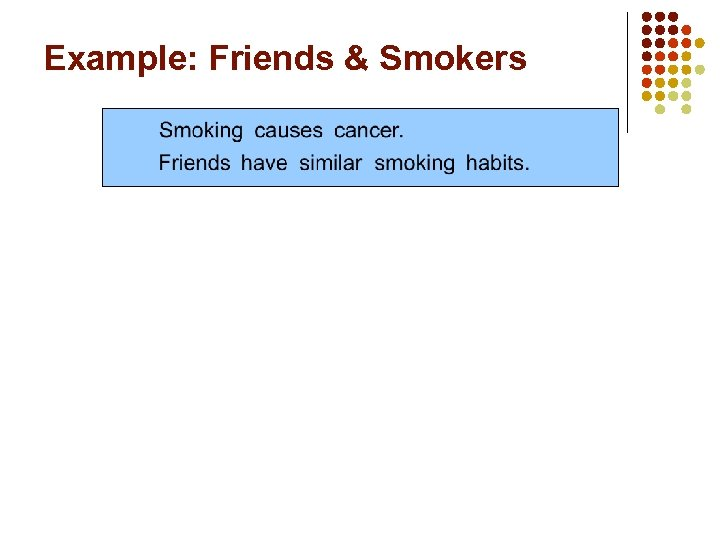Example: Friends & Smokers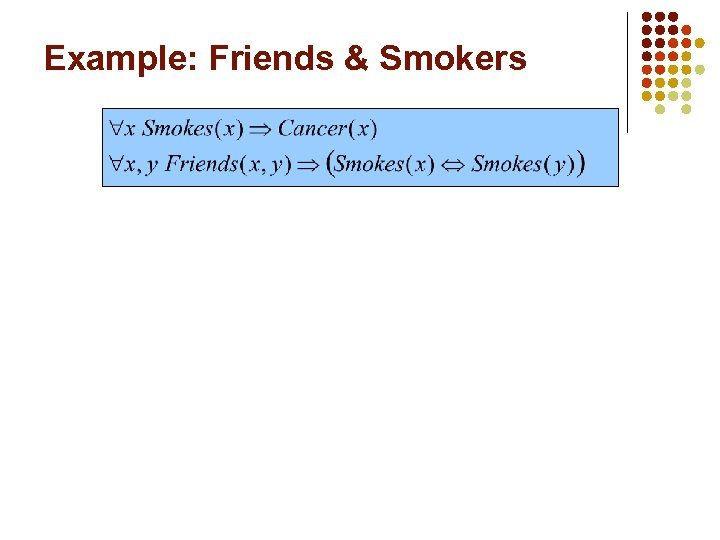Example: Friends & Smokers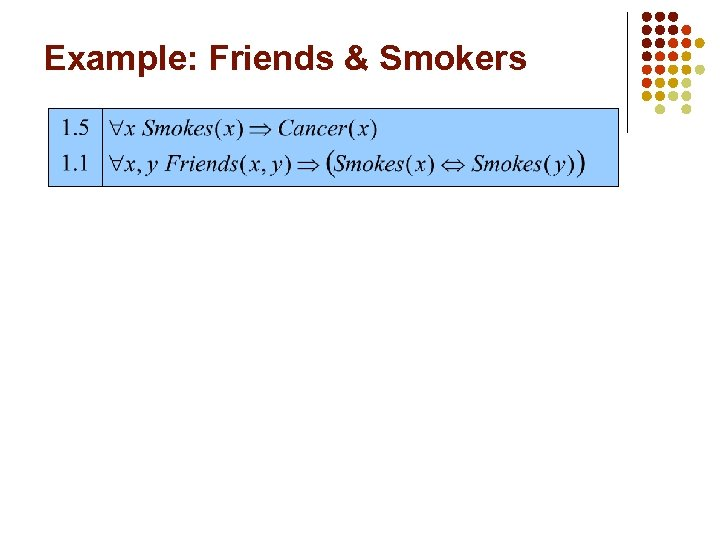Example: Friends & Smokers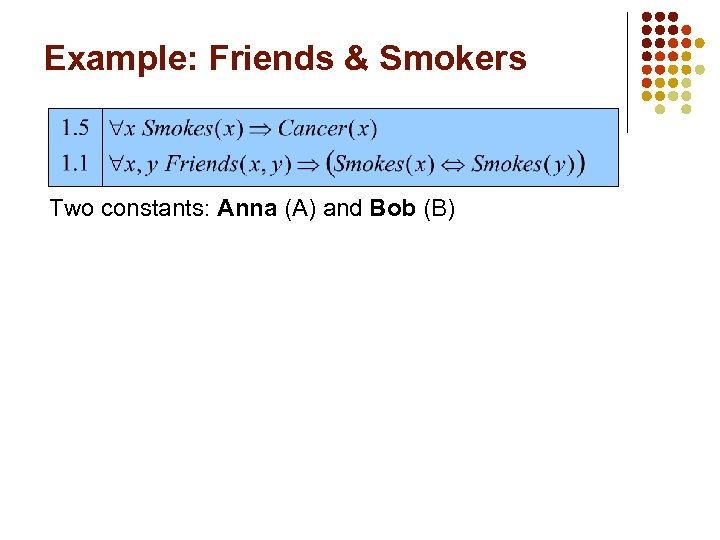Example: Friends & Smokers Two constants: Anna (A) and Bob (B)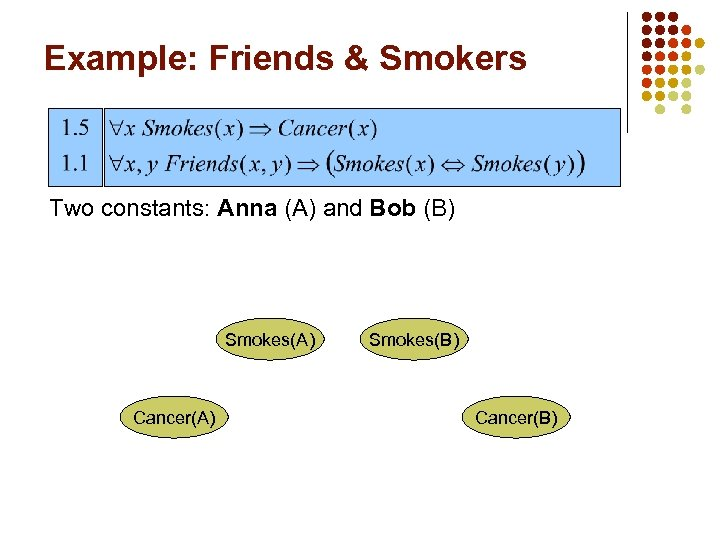Example: Friends & Smokers Two constants: Anna (A) and Bob (B) Smokes(A) Cancer(A) Smokes(B) Cancer(B)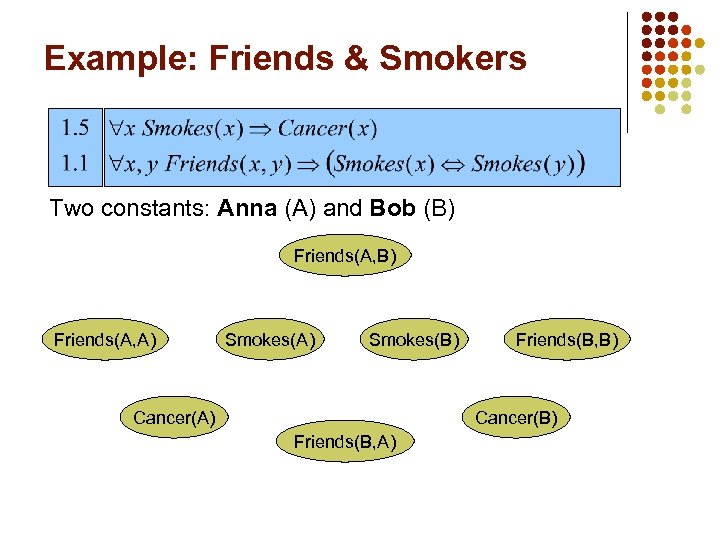Example: Friends & Smokers Two constants: Anna (A) and Bob (B) Friends(A, A) Smokes(B) Cancer(A) Friends(B, B) Cancer(B) Friends(B, A)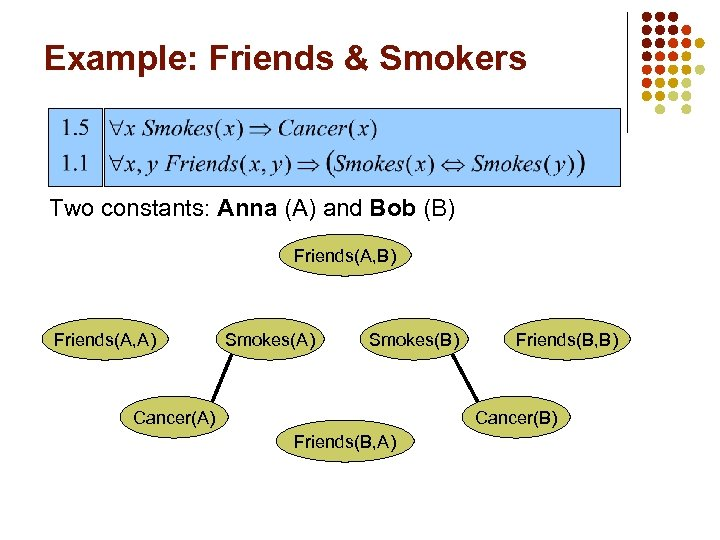Example: Friends & Smokers Two constants: Anna (A) and Bob (B) Friends(A, A) Smokes(B) Cancer(A) Friends(B, B) Cancer(B) Friends(B, A)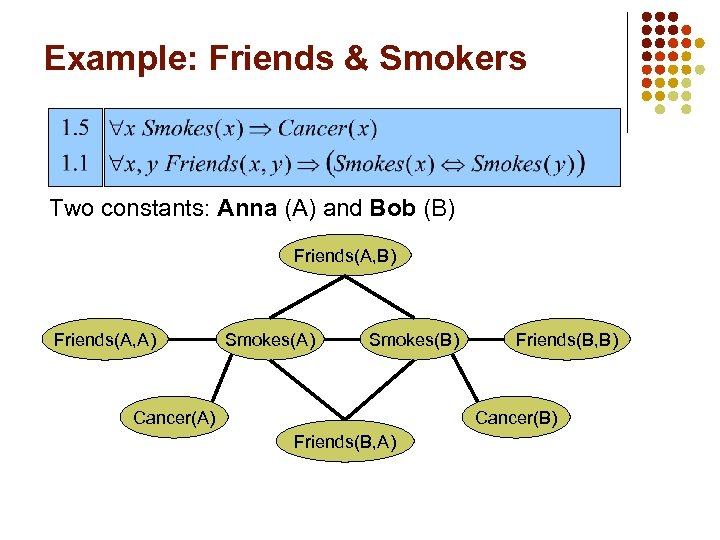Example: Friends & Smokers Two constants: Anna (A) and Bob (B) Friends(A, A) Smokes(B) Cancer(A) Friends(B, B) Cancer(B) Friends(B, A)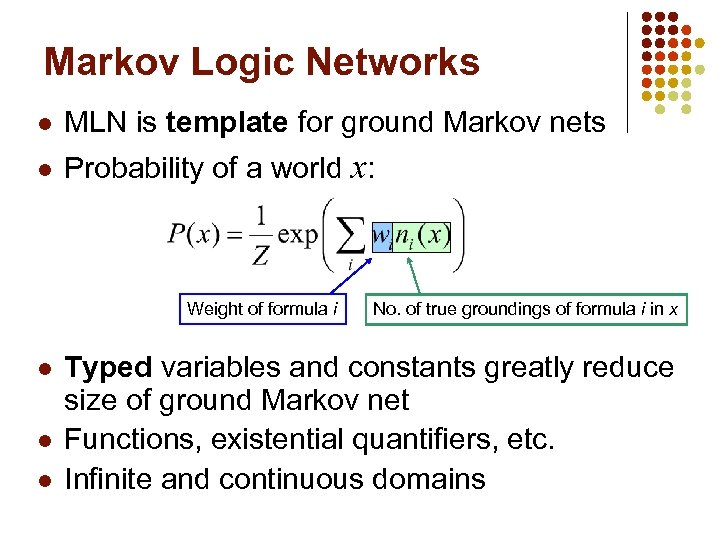Markov Logic Networks l MLN is template for ground Markov nets l Probability of a world x: Weight of formula i l l l No. of true groundings of formula i in x Typed variables and constants greatly reduce size of ground Markov net Functions, existential quantifiers, etc. Infinite and continuous domains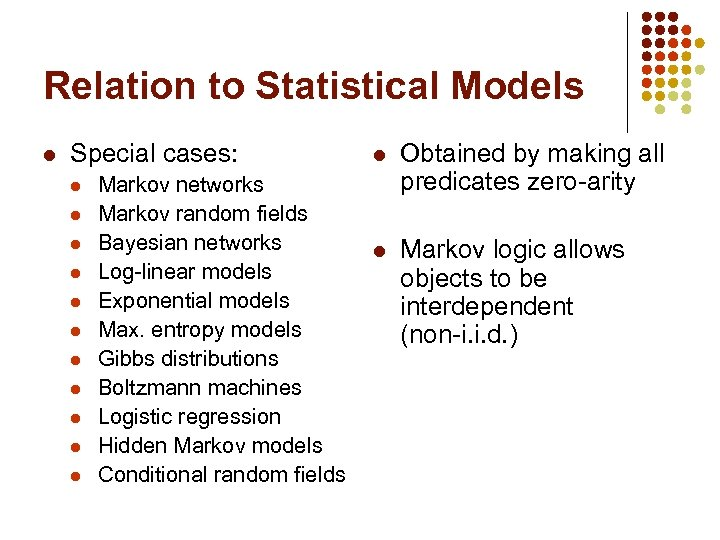Relation to Statistical Models l Special cases: l l l Markov networks Markov random fields Bayesian networks Log-linear models Exponential models Max. entropy models Gibbs distributions Boltzmann machines Logistic regression Hidden Markov models Conditional random fields l Obtained by making all predicates zero-arity l Markov logic allows objects to be interdependent (non-i. i. d. )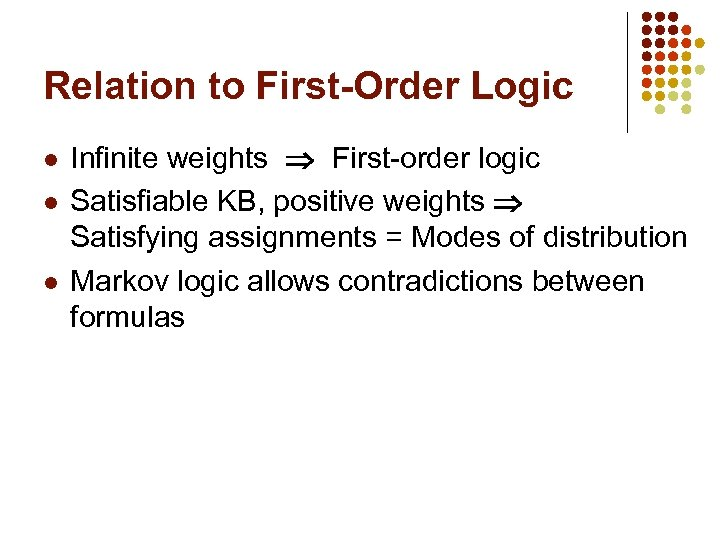Relation to First-Order Logic l l l Infinite weights First-order logic Satisfiable KB, positive weights Satisfying assignments = Modes of distribution Markov logic allows contradictions between formulas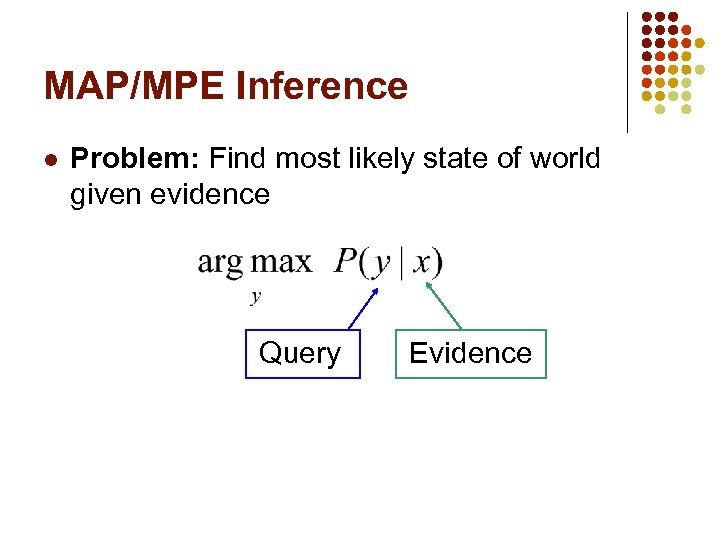MAP/MPE Inference l Problem: Find most likely state of world given evidence Query Evidence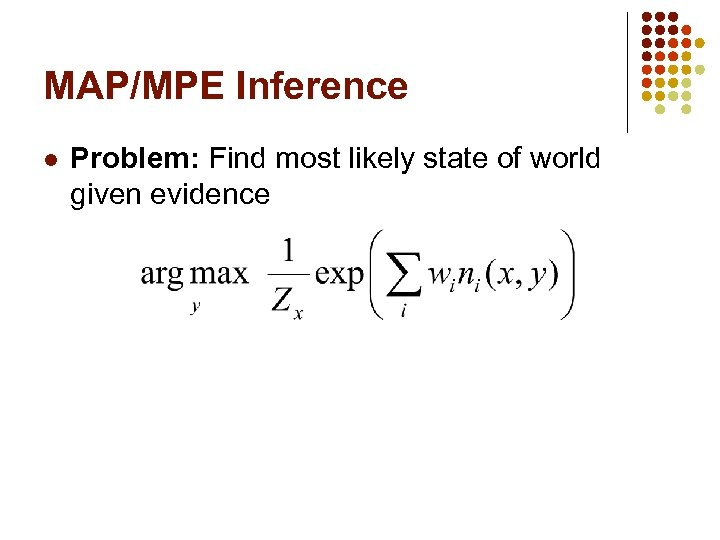MAP/MPE Inference l Problem: Find most likely state of world given evidence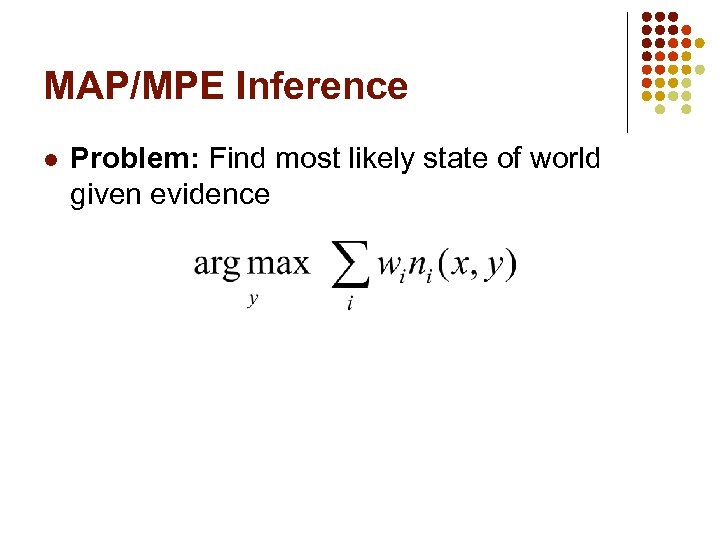MAP/MPE Inference l Problem: Find most likely state of world given evidence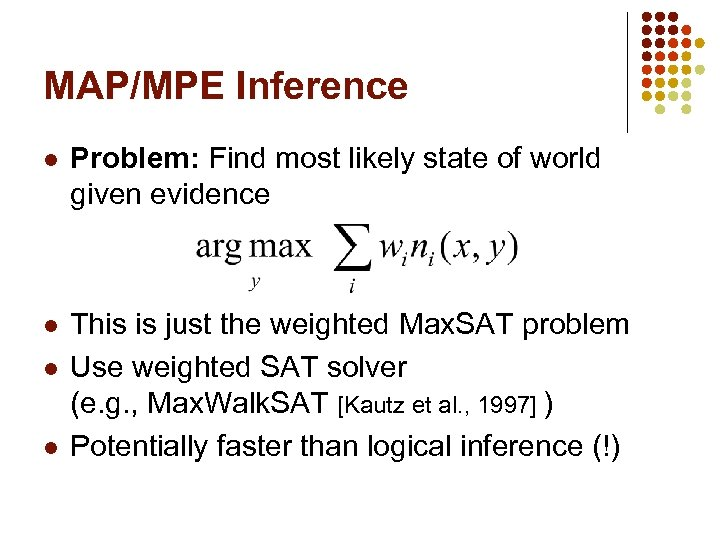MAP/MPE Inference l Problem: Find most likely state of world given evidence l This is just the weighted Max. SAT problem Use weighted SAT solver (e. g. , Max. Walk. SAT [Kautz et al. , 1997] ) Potentially faster than logical inference (!) l l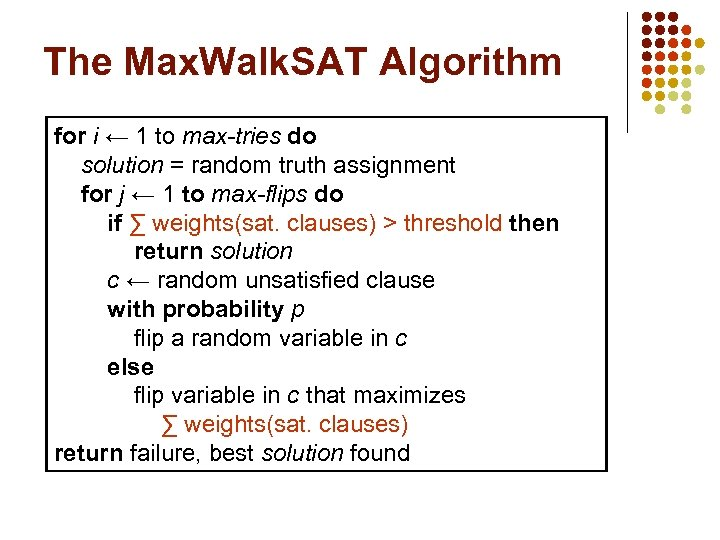The Max. Walk. SAT Algorithm for i ← 1 to max-tries do solution = random truth assignment for j ← 1 to max-flips do if ∑ weights(sat. clauses) > threshold then return solution c ← random unsatisfied clause with probability p flip a random variable in c else flip variable in c that maximizes ∑ weights(sat. clauses) return failure, best solution found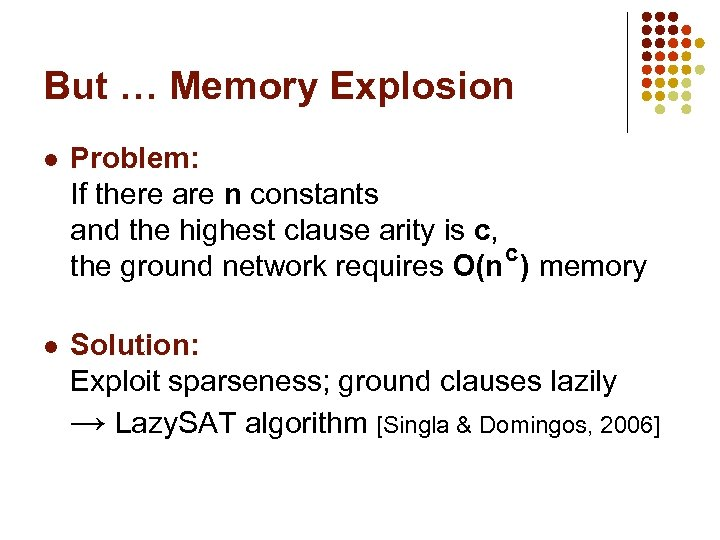But … Memory Explosion l Problem: If there are n constants and the highest clause arity is c, c the ground network requires O(n ) memory l Solution: Exploit sparseness; ground clauses lazily → Lazy. SAT algorithm [Singla & Domingos, 2006]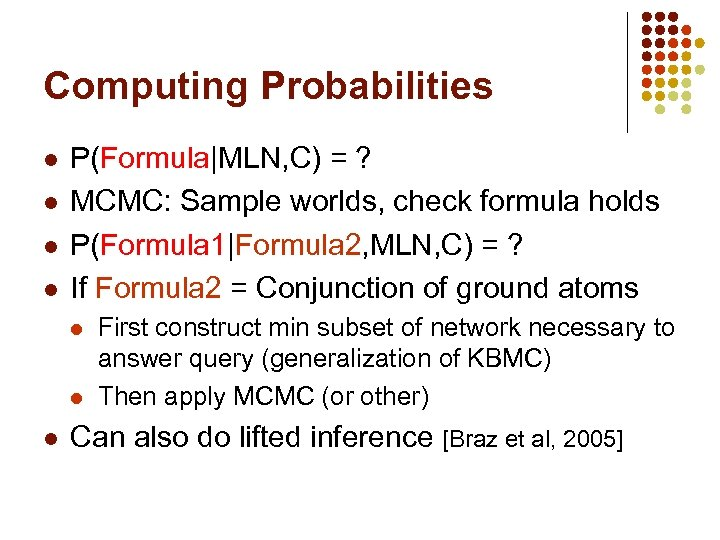Computing Probabilities l l P(Formula|MLN, C) = ? MCMC: Sample worlds, check formula holds P(Formula 1|Formula 2, MLN, C) = ? If Formula 2 = Conjunction of ground atoms l l l First construct min subset of network necessary to answer query (generalization of KBMC) Then apply MCMC (or other) Can also do lifted inference [Braz et al, 2005]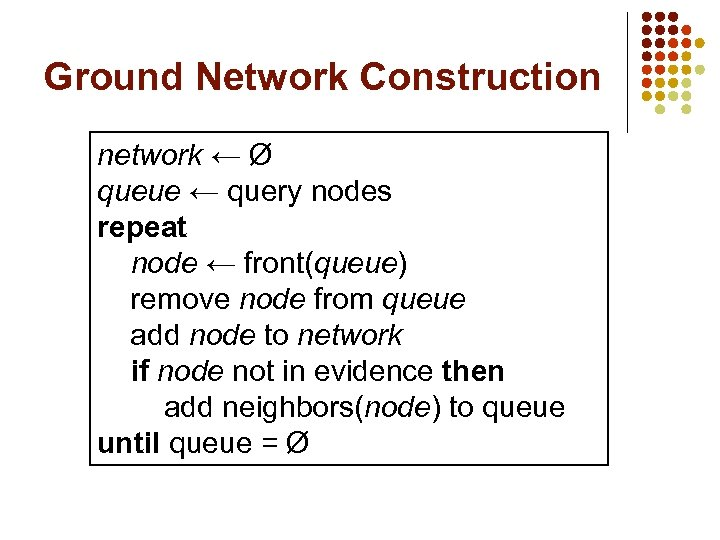Ground Network Construction network ← Ø queue ← query nodes repeat node ← front(queue) remove node from queue add node to network if node not in evidence then add neighbors(node) to queue until queue = Ø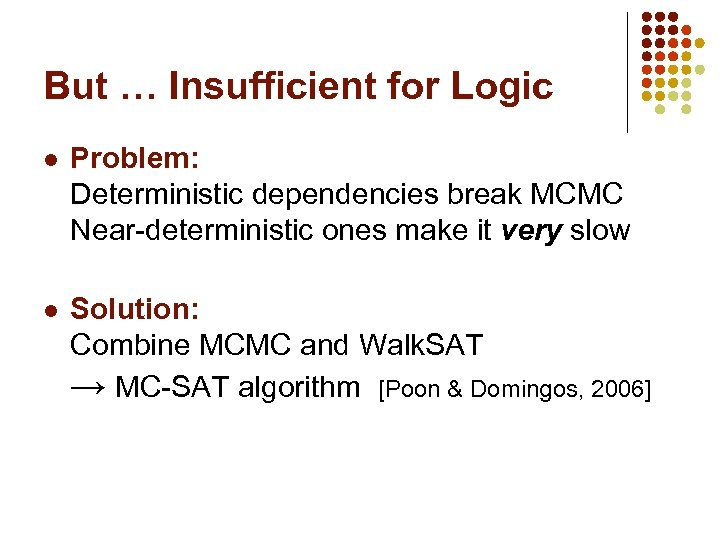But … Insufficient for Logic l Problem: Deterministic dependencies break MCMC Near-deterministic ones make it very slow l Solution: Combine MCMC and Walk. SAT → MC-SAT algorithm [Poon & Domingos, 2006]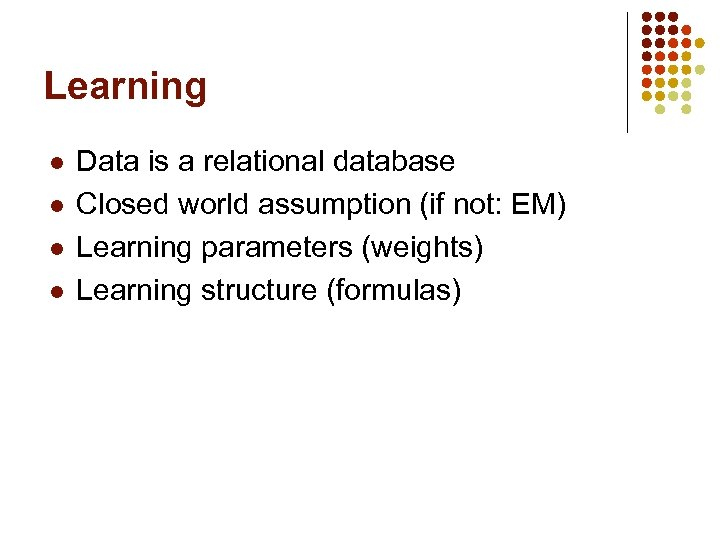Learning l l Data is a relational database Closed world assumption (if not: EM) Learning parameters (weights) Learning structure (formulas)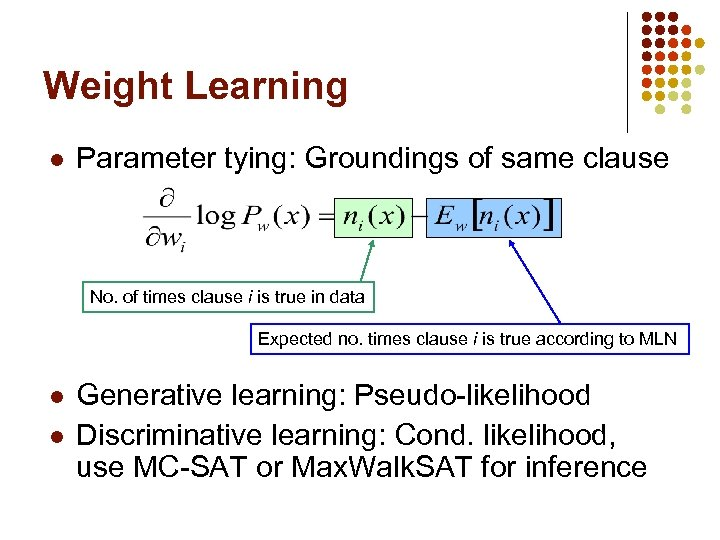Weight Learning l Parameter tying: Groundings of same clause No. of times clause i is true in data Expected no. times clause i is true according to MLN l l Generative learning: Pseudo-likelihood Discriminative learning: Cond. likelihood, use MC-SAT or Max. Walk. SAT for inference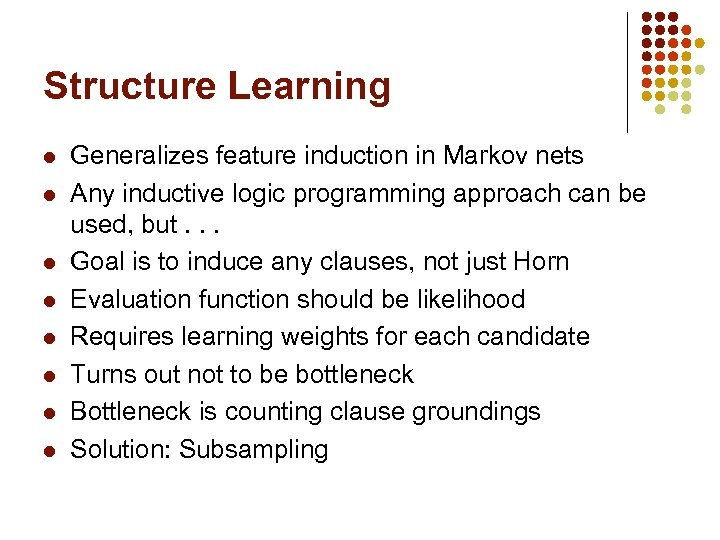Structure Learning l l l l Generalizes feature induction in Markov nets Any inductive logic programming approach can be used, but. . . Goal is to induce any clauses, not just Horn Evaluation function should be likelihood Requires learning weights for each candidate Turns out not to be bottleneck Bottleneck is counting clause groundings Solution: Subsampling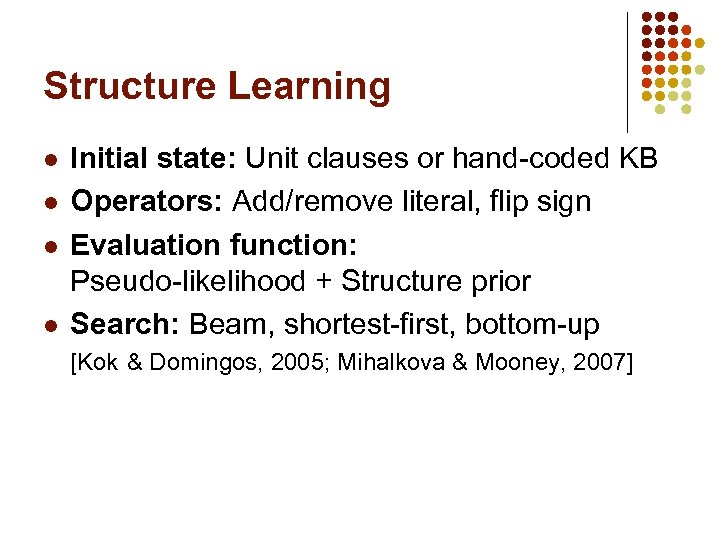Structure Learning l l Initial state: Unit clauses or hand-coded KB Operators: Add/remove literal, flip sign Evaluation function: Pseudo-likelihood + Structure prior Search: Beam, shortest-first, bottom-up [Kok & Domingos, 2005; Mihalkova & Mooney, 2007]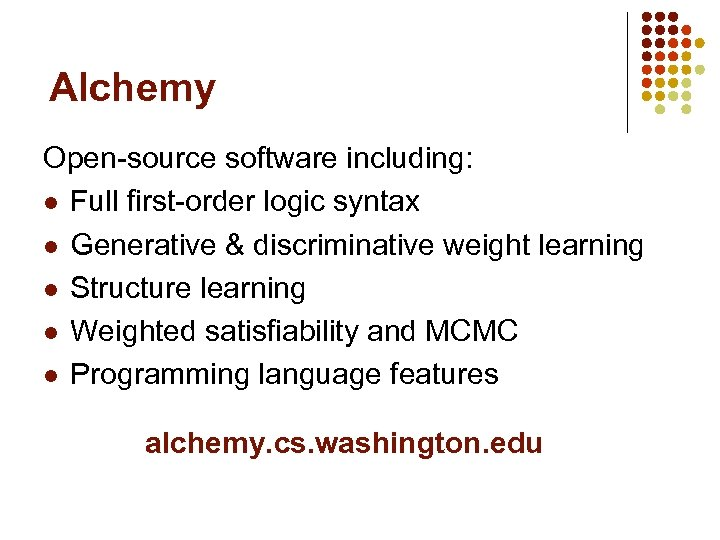Alchemy Open-source software including: l Full first-order logic syntax l Generative & discriminative weight learning l Structure learning l Weighted satisfiability and MCMC l Programming language features alchemy. cs. washington. edu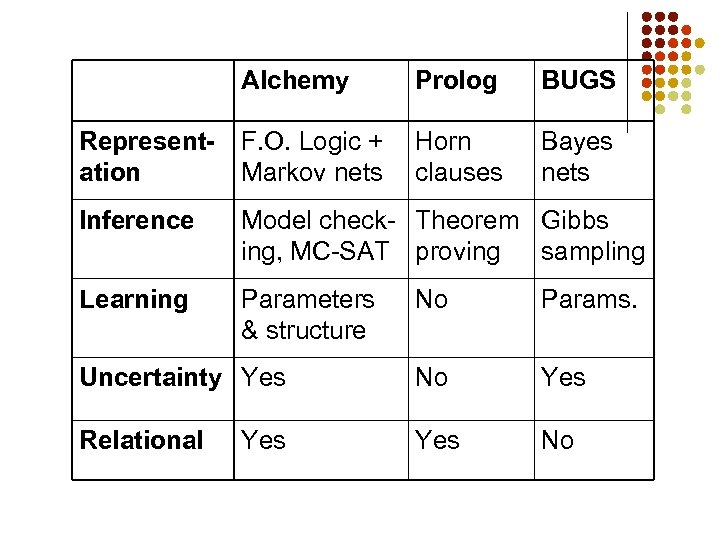Alchemy Prolog BUGS Representation F. O. Logic + Markov nets Horn clauses Bayes nets Inference Model check- Theorem Gibbs ing, MC-SAT proving sampling Learning Parameters & structure No Params. Uncertainty Yes No Yes Relational Yes No Yes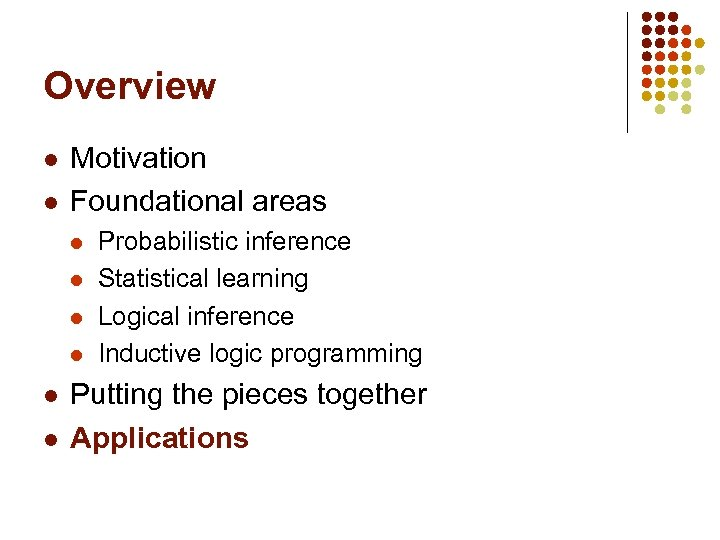Overview l l Motivation Foundational areas l l l Probabilistic inference Statistical learning Logical inference Inductive logic programming Putting the pieces together Applications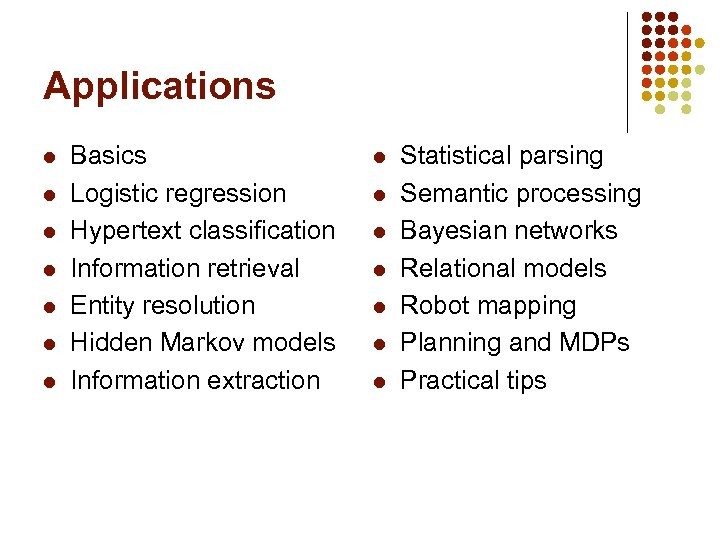Applications l l l l Basics Logistic regression Hypertext classification Information retrieval Entity resolution Hidden Markov models Information extraction l l l l Statistical parsing Semantic processing Bayesian networks Relational models Robot mapping Planning and MDPs Practical tips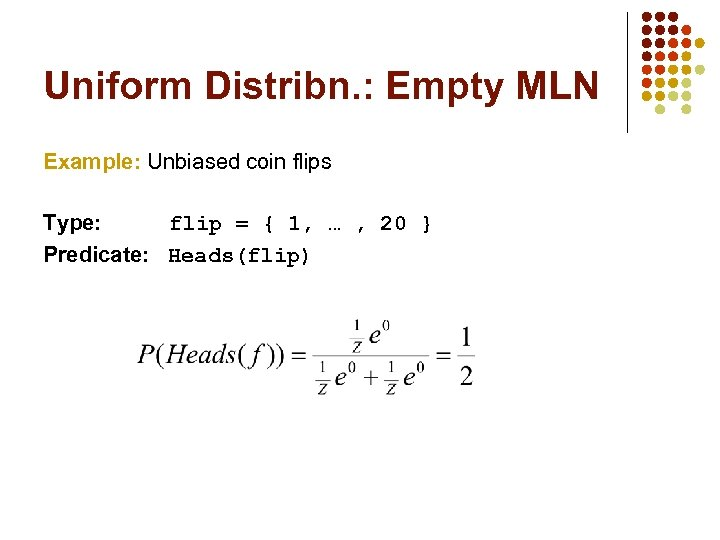Uniform Distribn. : Empty MLN Example: Unbiased coin flips Type: flip = { 1, … , 20 } Predicate: Heads(flip)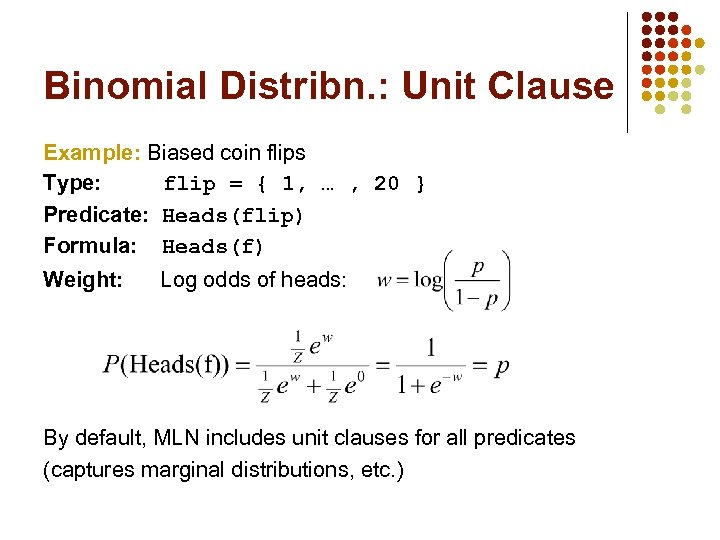Binomial Distribn. : Unit Clause Example: Biased coin flips Type: flip = { 1, … , 20 } Predicate: Heads(flip) Formula: Heads(f) Weight: Log odds of heads: By default, MLN includes unit clauses for all predicates (captures marginal distributions, etc. )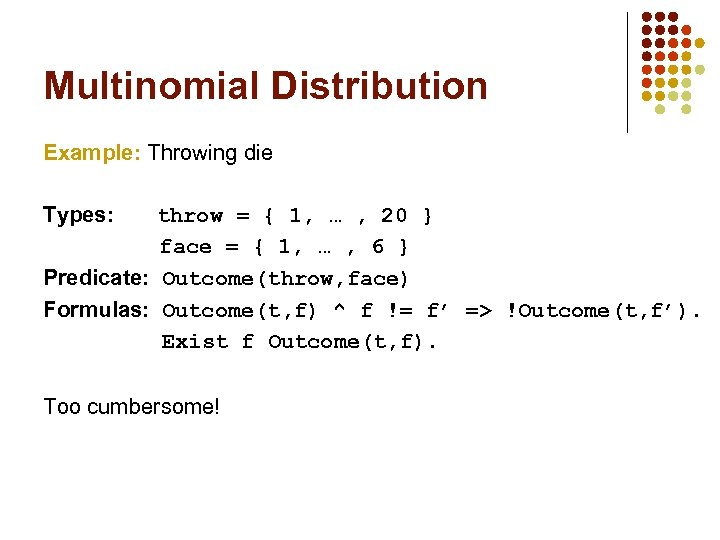Multinomial Distribution Example: Throwing die Types: throw = { 1, … , 20 } face = { 1, … , 6 } Predicate: Outcome(throw, face) Formulas: Outcome(t, f) ^ f != f’ => !Outcome(t, f’). Exist f Outcome(t, f). Too cumbersome!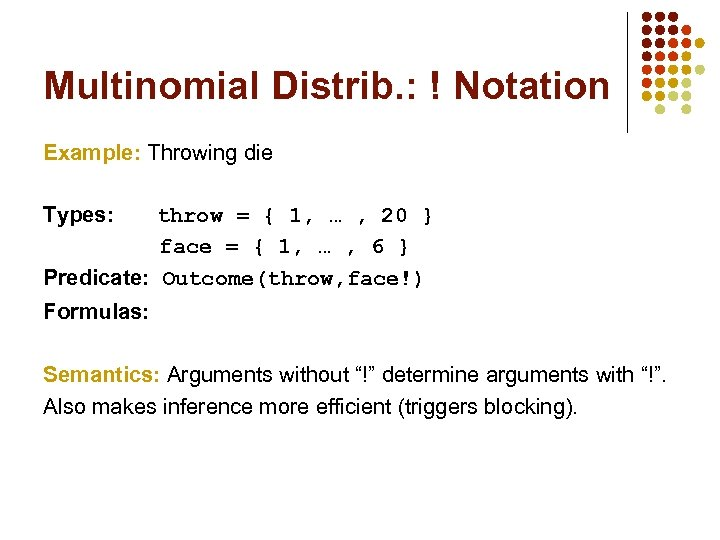Multinomial Distrib. : ! Notation Example: Throwing die Types: throw = { 1, … , 20 } face = { 1, … , 6 } Predicate: Outcome(throw, face!) Formulas: Semantics: Arguments without “!” determine arguments with “!”. Also makes inference more efficient (triggers blocking).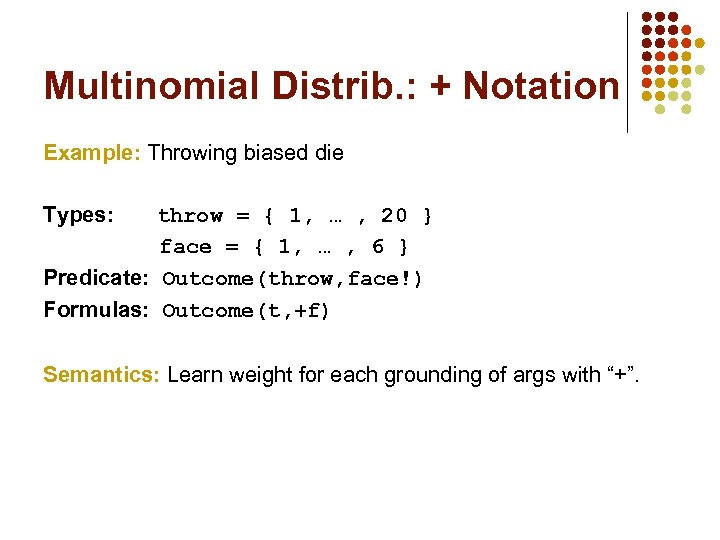Multinomial Distrib. : + Notation Example: Throwing biased die Types: throw = { 1, … , 20 } face = { 1, … , 6 } Predicate: Outcome(throw, face!) Formulas: Outcome(t, +f) Semantics: Learn weight for each grounding of args with “+”.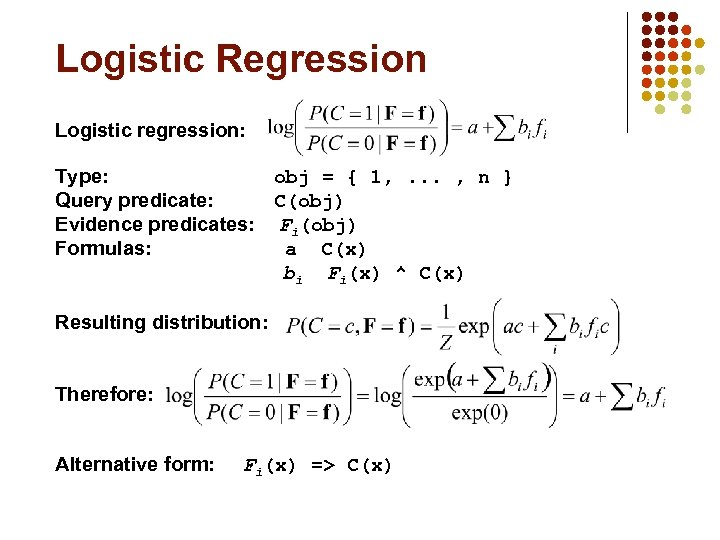Logistic Regression Logistic regression: Type: obj = { 1, . . . , n } Query predicate: C(obj) Evidence predicates: Fi(obj) Formulas: a C(x) bi Fi(x) ^ C(x) Resulting distribution: Therefore: Alternative form: Fi(x) => C(x)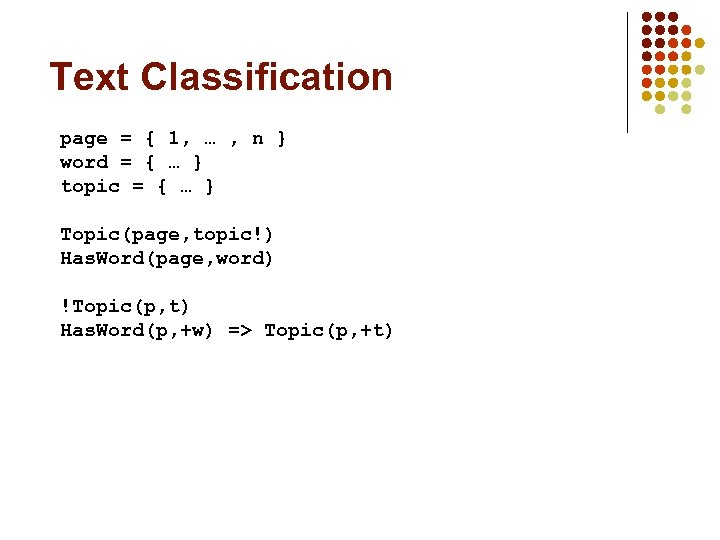Text Classification page = { 1, … , n } word = { … } topic = { … } Topic(page, topic!) Has. Word(page, word) !Topic(p, t) Has. Word(p, +w) => Topic(p, +t)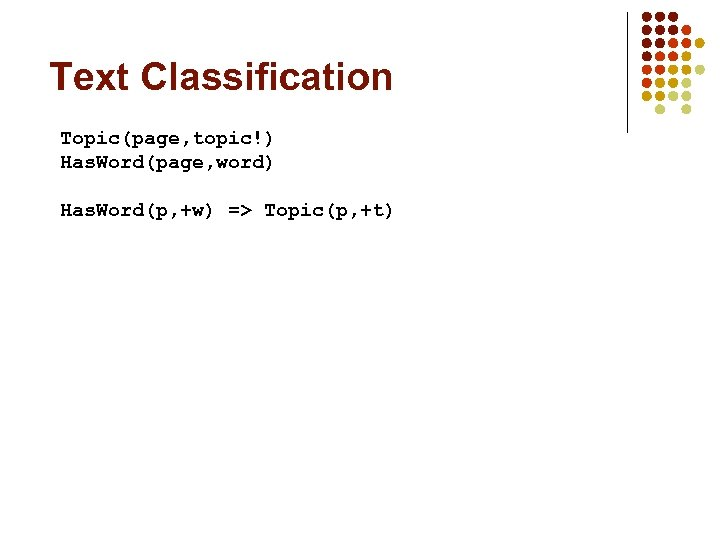Text Classification Topic(page, topic!) Has. Word(page, word) Has. Word(p, +w) => Topic(p, +t)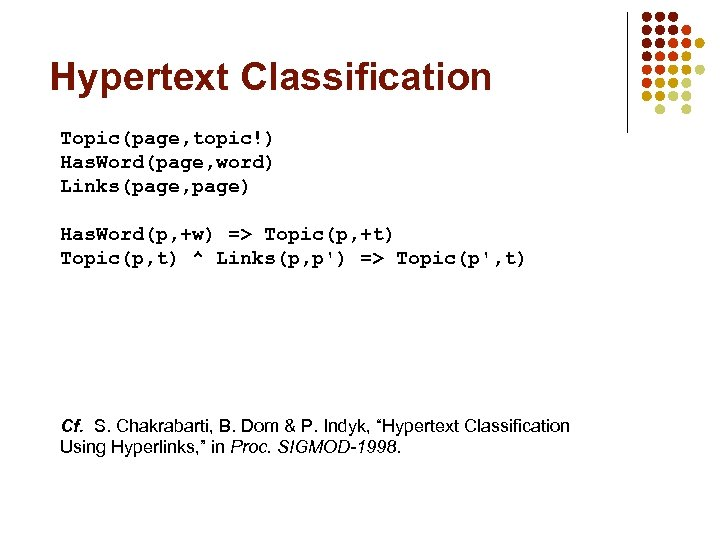Hypertext Classification Topic(page, topic!) Has. Word(page, word) Links(page, page) Has. Word(p, +w) => Topic(p, +t) Topic(p, t) ^ Links(p, p') => Topic(p', t) Cf. S. Chakrabarti, B. Dom & P. Indyk, “Hypertext Classification Using Hyperlinks, ” in Proc. SIGMOD-1998.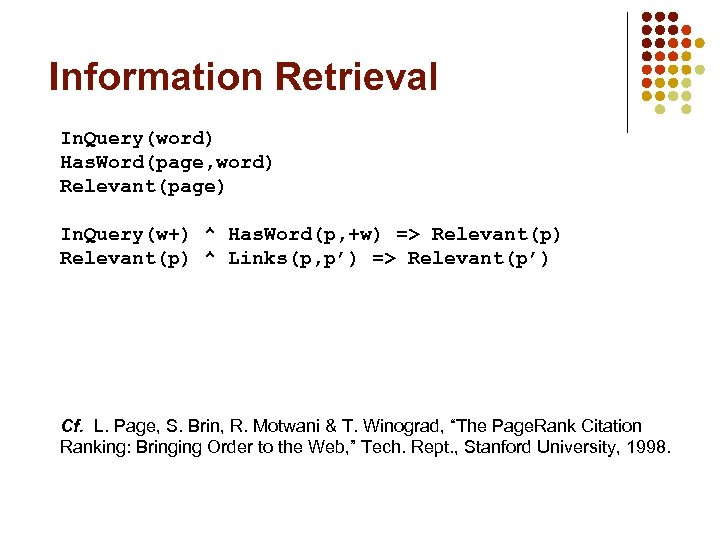Information Retrieval In. Query(word) Has. Word(page, word) Relevant(page) In. Query(w+) ^ Has. Word(p, +w) => Relevant(p) ^ Links(p, p’) => Relevant(p’) Cf. L. Page, S. Brin, R. Motwani & T. Winograd, “The Page. Rank Citation Ranking: Bringing Order to the Web, ” Tech. Rept. , Stanford University, 1998.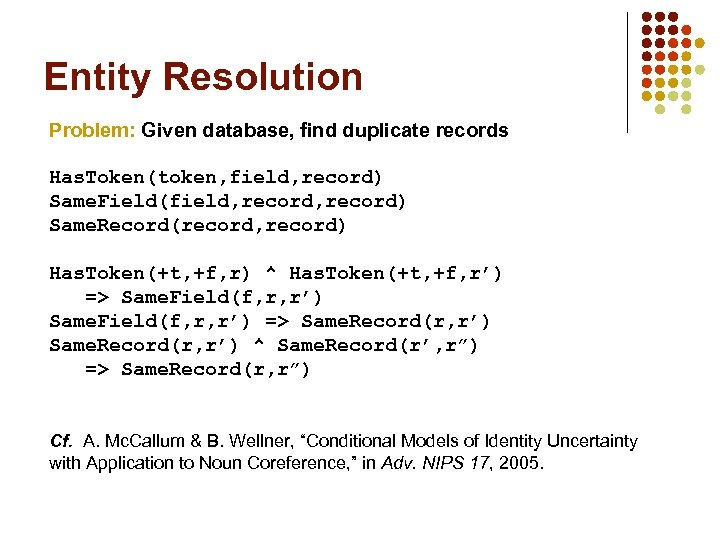Entity Resolution Problem: Given database, find duplicate records Has. Token(token, field, record) Same. Field(field, record) Same. Record(record, record) Has. Token(+t, +f, r) ^ Has. Token(+t, +f, r’) => Same. Field(f, r, r’) => Same. Record(r, r’) ^ Same. Record(r’, r”) => Same. Record(r, r”) Cf. A. Mc. Callum & B. Wellner, “Conditional Models of Identity Uncertainty with Application to Noun Coreference, ” in Adv. NIPS 17, 2005.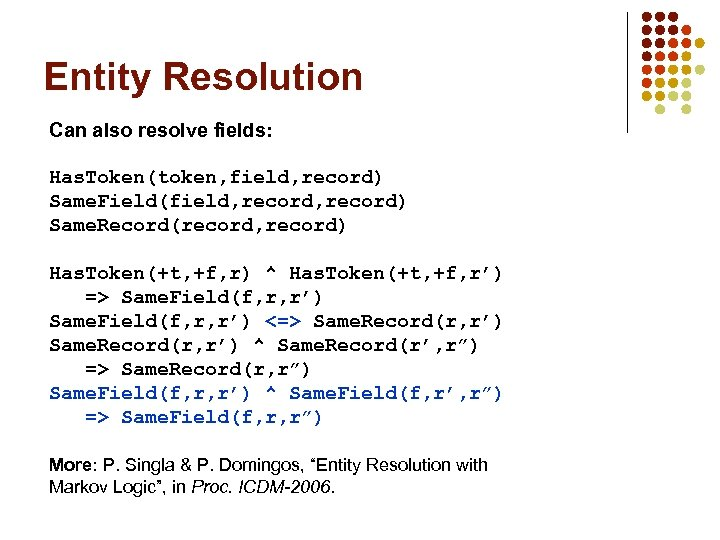Entity Resolution Can also resolve fields: Has. Token(token, field, record) Same. Field(field, record) Same. Record(record, record) Has. Token(+t, +f, r) ^ Has. Token(+t, +f, r’) => Same. Field(f, r, r’) <=> Same. Record(r, r’) ^ Same. Record(r’, r”) => Same. Record(r, r”) Same. Field(f, r, r’) ^ Same. Field(f, r’, r”) => Same. Field(f, r, r”) More: P. Singla & P. Domingos, “Entity Resolution with Markov Logic”, in Proc. ICDM-2006.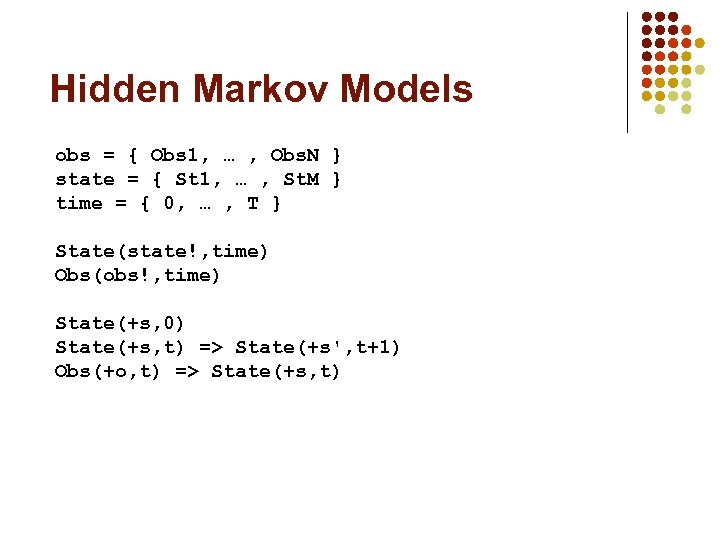Hidden Markov Models obs = { Obs 1, … , Obs. N } state = { St 1, … , St. M } time = { 0, … , T } State(state!, time) Obs(obs!, time) State(+s, 0) State(+s, t) => State(+s', t+1) Obs(+o, t) => State(+s, t)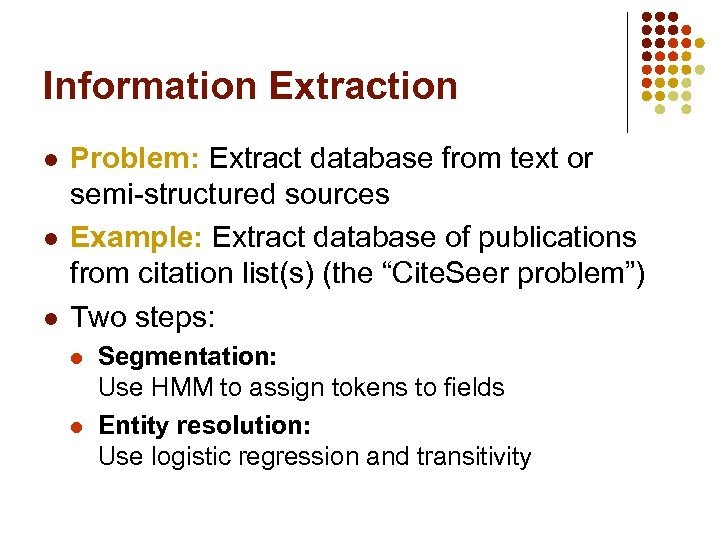Information Extraction l l l Problem: Extract database from text or semi-structured sources Example: Extract database of publications from citation list(s) (the “Cite. Seer problem”) Two steps: l l Segmentation: Use HMM to assign tokens to fields Entity resolution: Use logistic regression and transitivity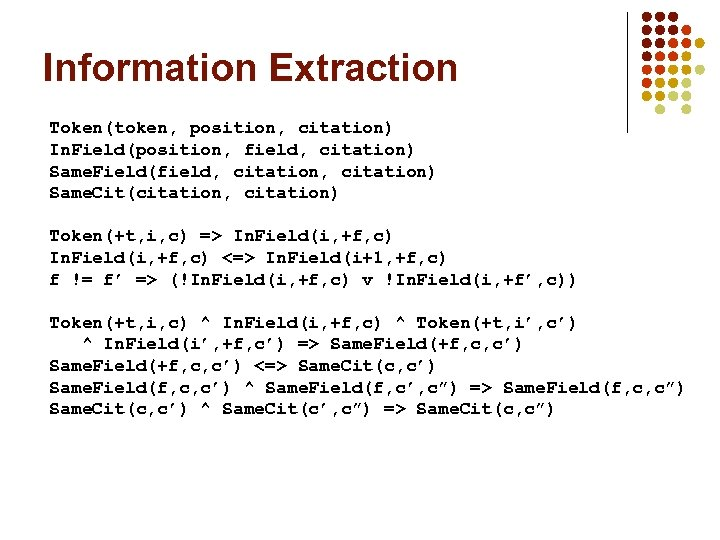Information Extraction Token(token, position, citation) In. Field(position, field, citation) Same. Field(field, citation) Same. Cit(citation, citation) Token(+t, i, c) => In. Field(i, +f, c) <=> In. Field(i+1, +f, c) f != f’ => (!In. Field(i, +f, c) v !In. Field(i, +f’, c)) Token(+t, i, c) ^ In. Field(i, +f, c) ^ Token(+t, i’, c’) ^ In. Field(i’, +f, c’) => Same. Field(+f, c, c’) <=> Same. Cit(c, c’) Same. Field(f, c, c’) ^ Same. Field(f, c’, c”) => Same. Field(f, c, c”) Same. Cit(c, c’) ^ Same. Cit(c’, c”) => Same. Cit(c, c”)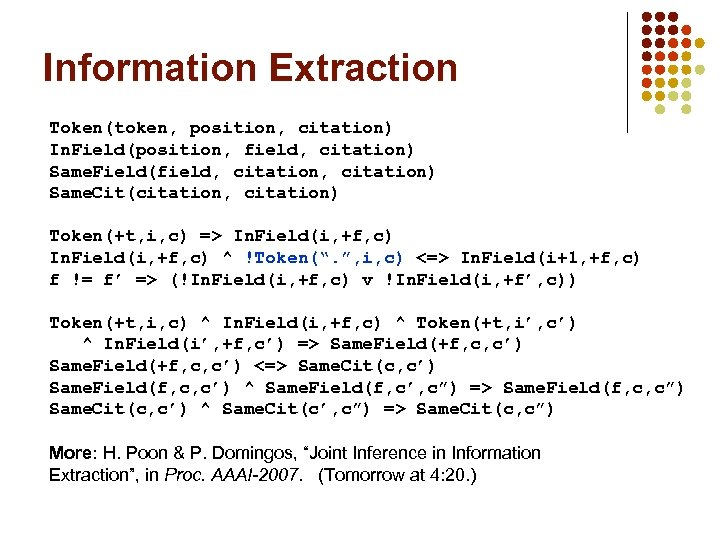Information Extraction Token(token, position, citation) In. Field(position, field, citation) Same. Field(field, citation) Same. Cit(citation, citation) Token(+t, i, c) => In. Field(i, +f, c) ^ !Token(“. ”, i, c) <=> In. Field(i+1, +f, c) f != f’ => (!In. Field(i, +f, c) v !In. Field(i, +f’, c)) Token(+t, i, c) ^ In. Field(i, +f, c) ^ Token(+t, i’, c’) ^ In. Field(i’, +f, c’) => Same. Field(+f, c, c’) <=> Same. Cit(c, c’) Same. Field(f, c, c’) ^ Same. Field(f, c’, c”) => Same. Field(f, c, c”) Same. Cit(c, c’) ^ Same. Cit(c’, c”) => Same. Cit(c, c”) More: H. Poon & P. Domingos, “Joint Inference in Information Extraction”, in Proc. AAAI-2007. (Tomorrow at 4: 20. )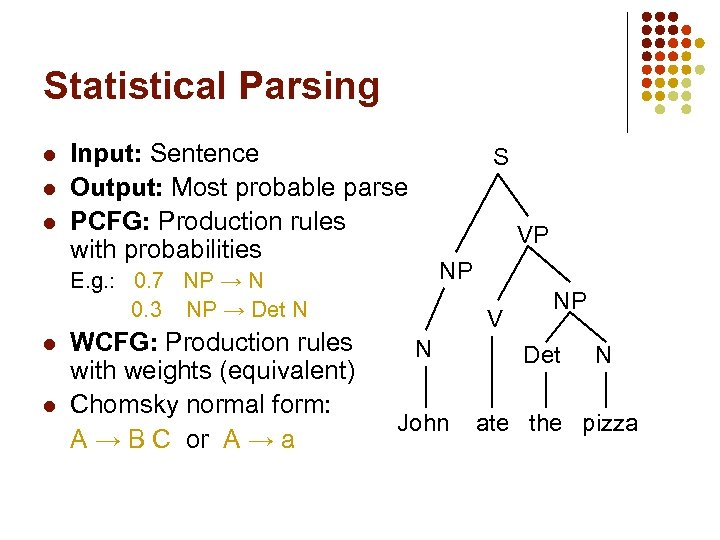Statistical Parsing l l l Input: Sentence Output: Most probable parse PCFG: Production rules with probabilities S VP NP E. g. : 0. 7 NP → N 0. 3 NP → Det N l l WCFG: Production rules with weights (equivalent) Chomsky normal form: A → B C or A → a V N John NP Det N ate the pizza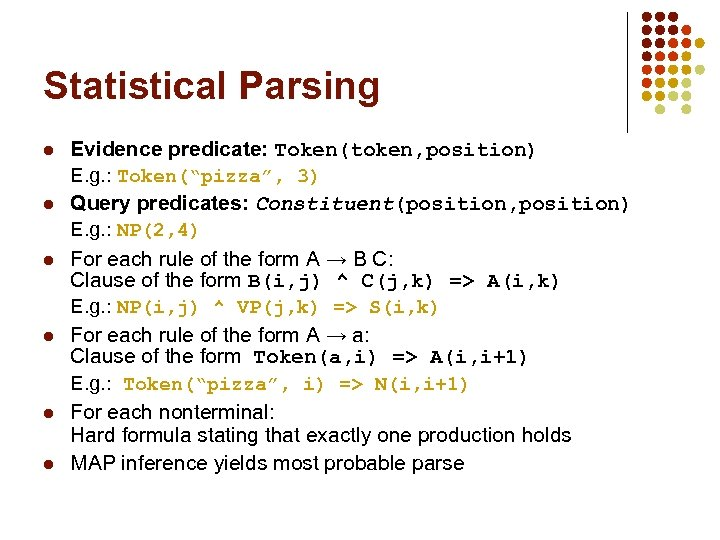Statistical Parsing l l l Evidence predicate: Token(token, position) E. g. : Token(“pizza”, 3) Query predicates: Constituent(position, position) E. g. : NP(2, 4) For each rule of the form A → B C: Clause of the form B(i, j) ^ C(j, k) => A(i, k) E. g. : NP(i, j) ^ VP(j, k) => S(i, k) For each rule of the form A → a: Clause of the form Token(a, i) => A(i, i+1) E. g. : Token(“pizza”, i) => N(i, i+1) For each nonterminal: Hard formula stating that exactly one production holds MAP inference yields most probable parse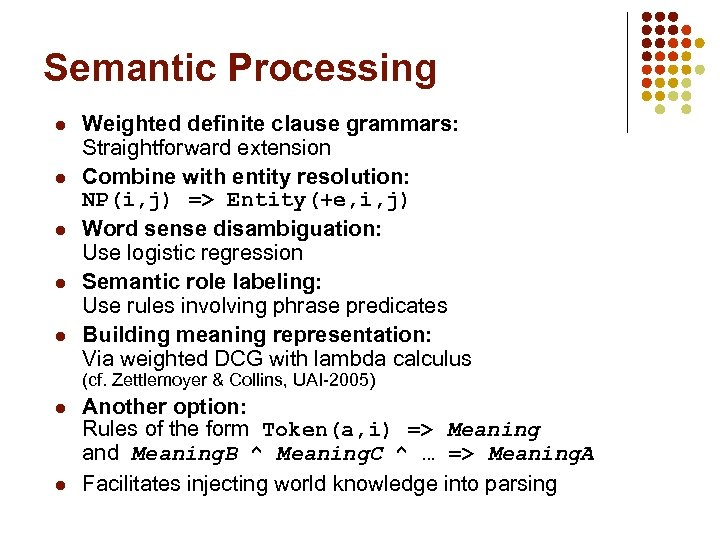Semantic Processing l l l Weighted definite clause grammars: Straightforward extension Combine with entity resolution: NP(i, j) => Entity(+e, i, j) Word sense disambiguation: Use logistic regression Semantic role labeling: Use rules involving phrase predicates Building meaning representation: Via weighted DCG with lambda calculus (cf. Zettlemoyer & Collins, UAI-2005) l l Another option: Rules of the form Token(a, i) => Meaning and Meaning. B ^ Meaning. C ^ … => Meaning. A Facilitates injecting world knowledge into parsing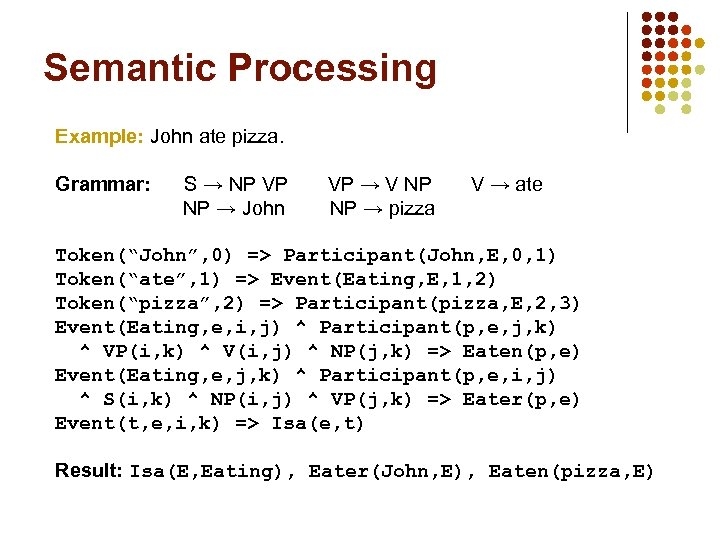Semantic Processing Example: John ate pizza. Grammar: S → NP VP NP → John VP → V NP NP → pizza V → ate Token(“John”, 0) => Participant(John, E, 0, 1) Token(“ate”, 1) => Event(Eating, E, 1, 2) Token(“pizza”, 2) => Participant(pizza, E, 2, 3) Event(Eating, e, i, j) ^ Participant(p, e, j, k) ^ VP(i, k) ^ V(i, j) ^ NP(j, k) => Eaten(p, e) Event(Eating, e, j, k) ^ Participant(p, e, i, j) ^ S(i, k) ^ NP(i, j) ^ VP(j, k) => Eater(p, e) Event(t, e, i, k) => Isa(e, t) Result: Isa(E, Eating), Eater(John, E), Eaten(pizza, E)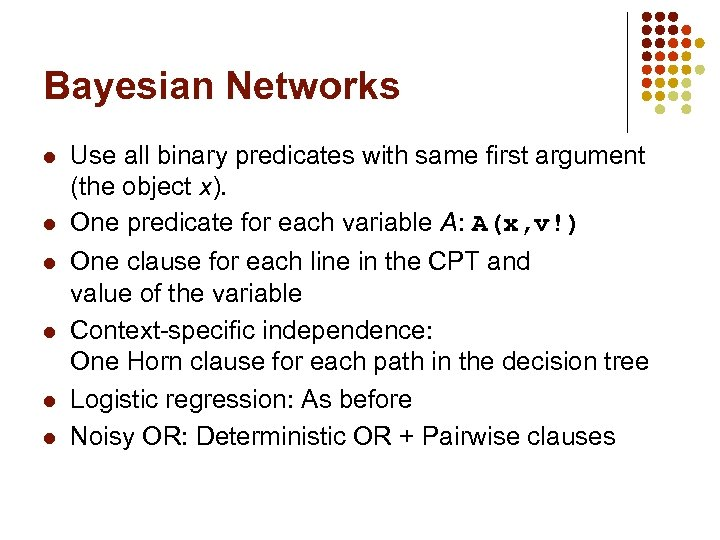Bayesian Networks l l l Use all binary predicates with same first argument (the object x). One predicate for each variable A: A(x, v!) One clause for each line in the CPT and value of the variable Context-specific independence: One Horn clause for each path in the decision tree Logistic regression: As before Noisy OR: Deterministic OR + Pairwise clauses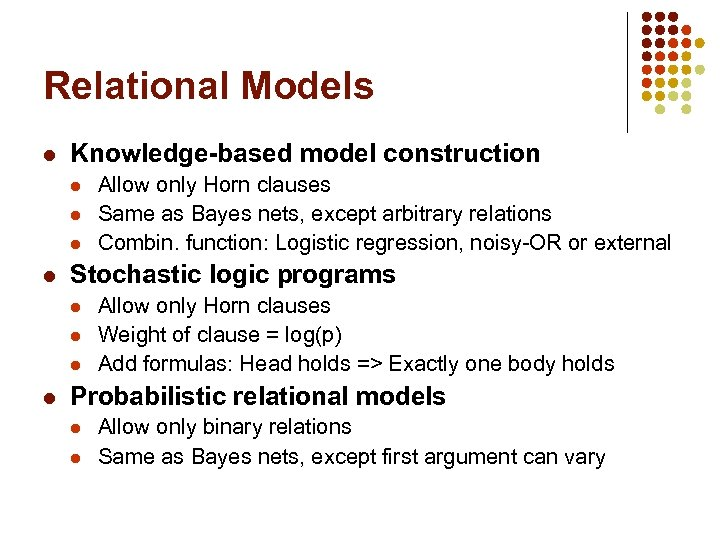Relational Models l Knowledge-based model construction l l Stochastic logic programs l l Allow only Horn clauses Same as Bayes nets, except arbitrary relations Combin. function: Logistic regression, noisy-OR or external Allow only Horn clauses Weight of clause = log(p) Add formulas: Head holds => Exactly one body holds Probabilistic relational models l l Allow only binary relations Same as Bayes nets, except first argument can vary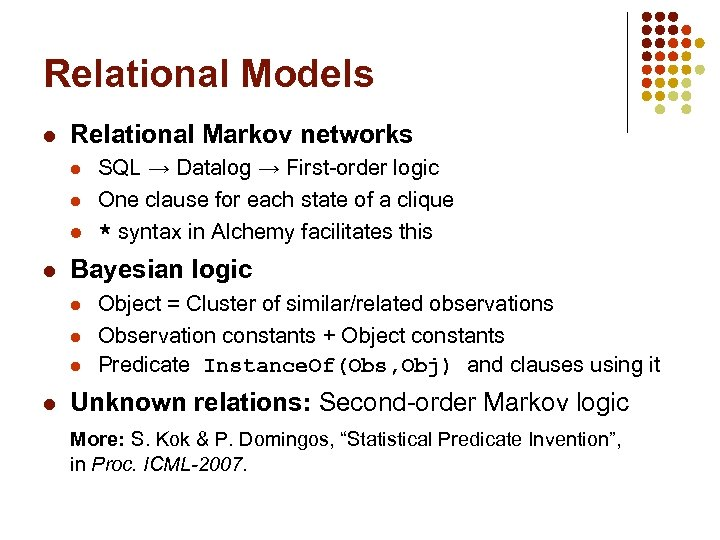Relational Models l Relational Markov networks l l Bayesian logic l l SQL → Datalog → First-order logic One clause for each state of a clique * syntax in Alchemy facilitates this Object = Cluster of similar/related observations Observation constants + Object constants Predicate Instance. Of(Obs, Obj) and clauses using it Unknown relations: Second-order Markov logic More: S. Kok & P. Domingos, “Statistical Predicate Invention”, in Proc. ICML-2007.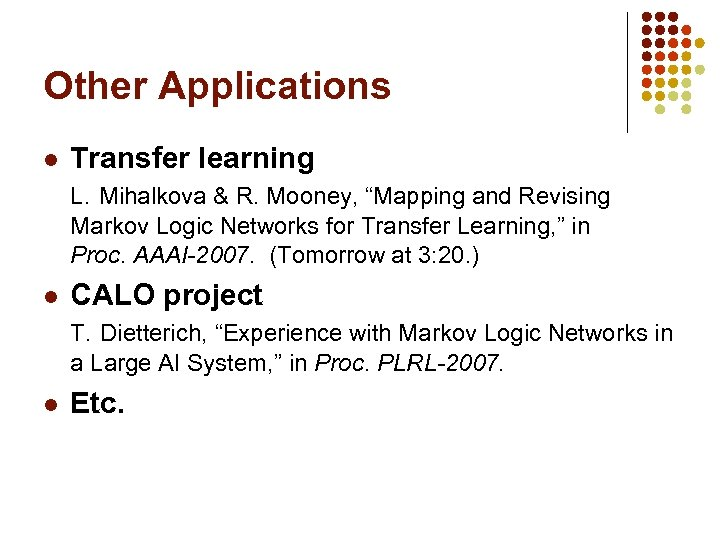Other Applications l Transfer learning L. Mihalkova & R. Mooney, “Mapping and Revising Markov Logic Networks for Transfer Learning, ” in Proc. AAAI-2007. (Tomorrow at 3: 20. ) l CALO project T. Dietterich, “Experience with Markov Logic Networks in a Large AI System, ” in Proc. PLRL-2007. l Etc.